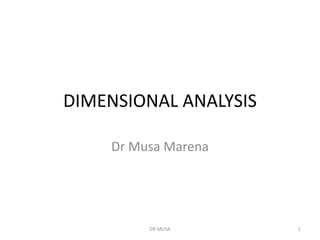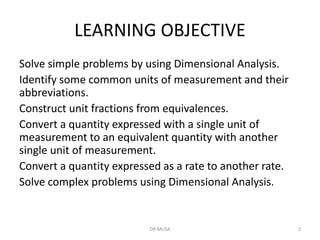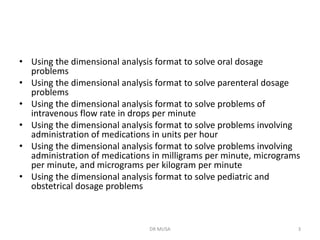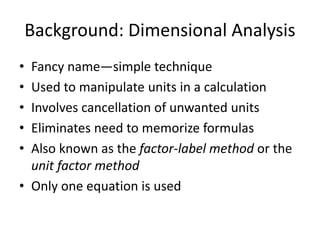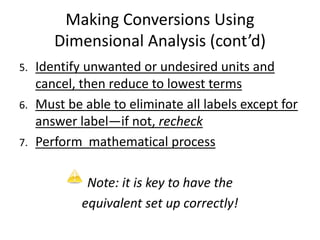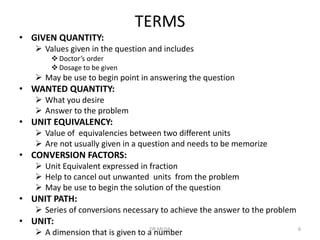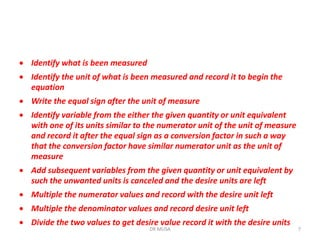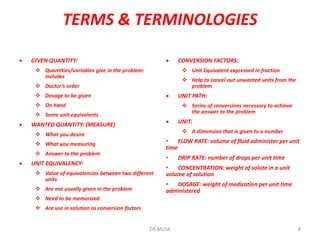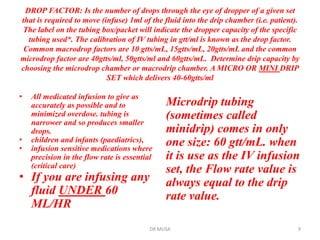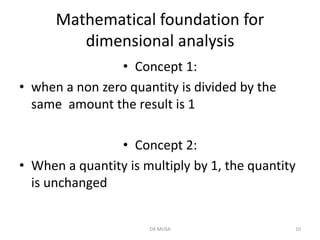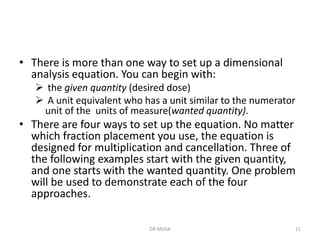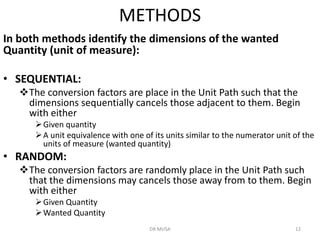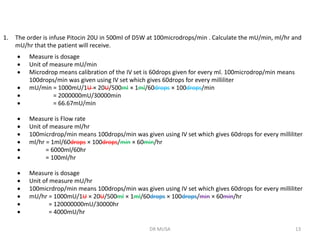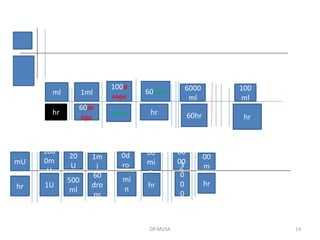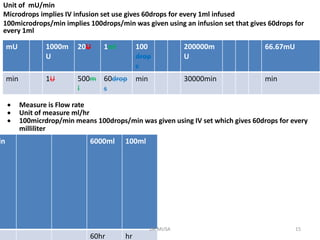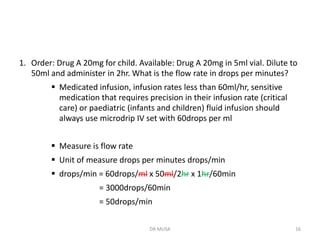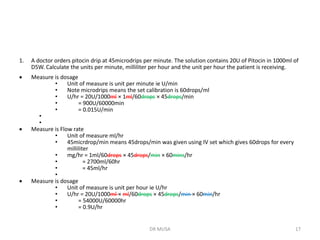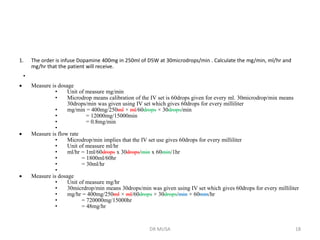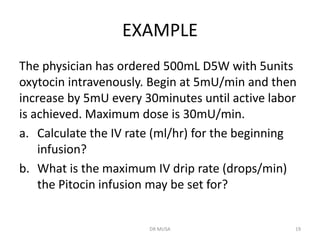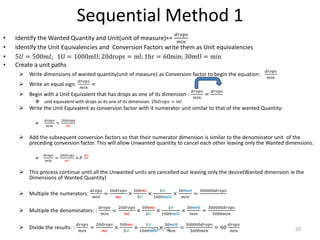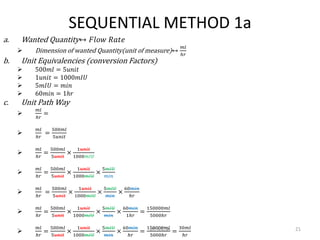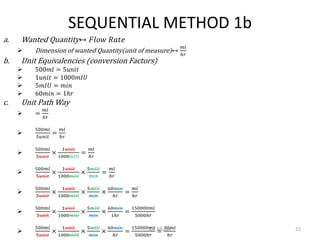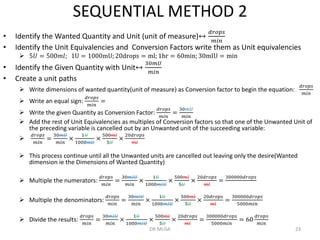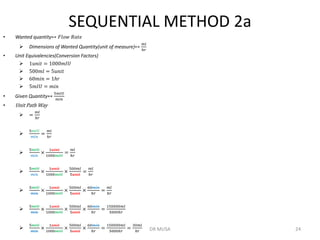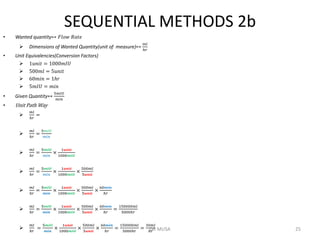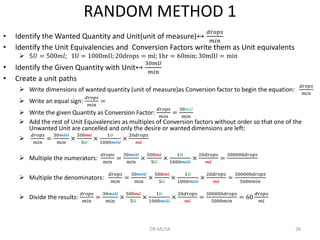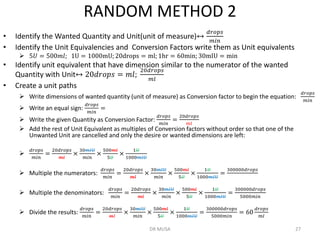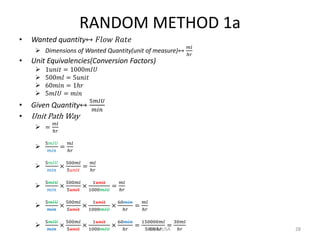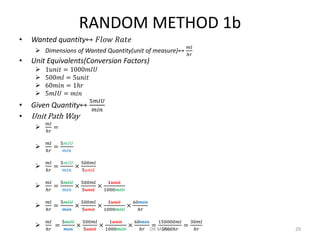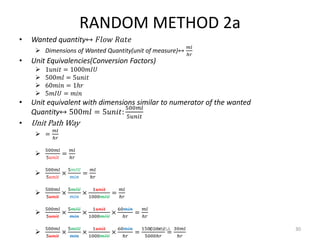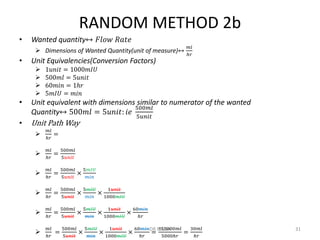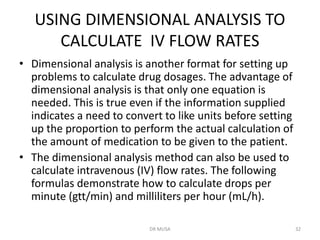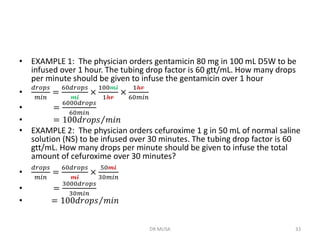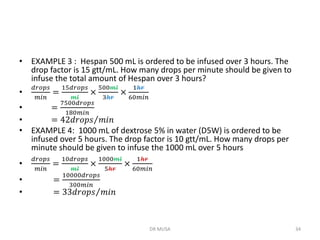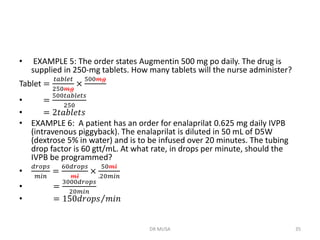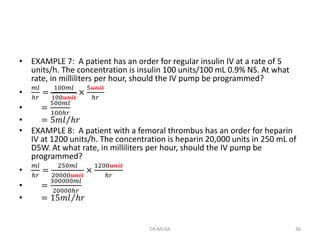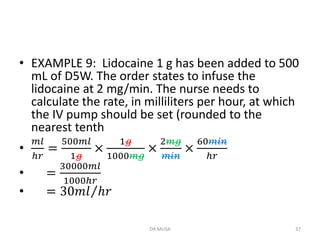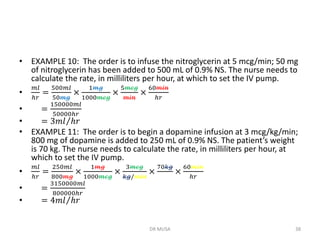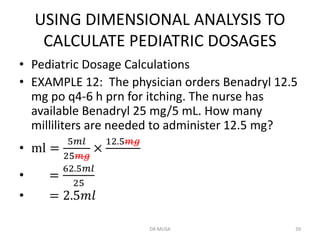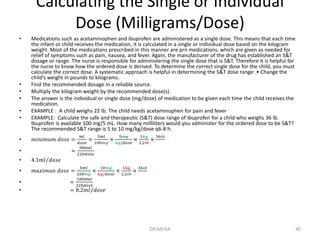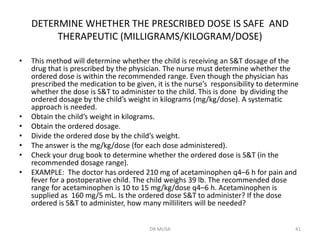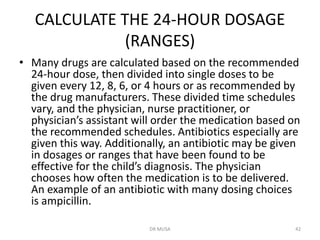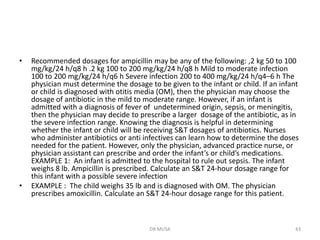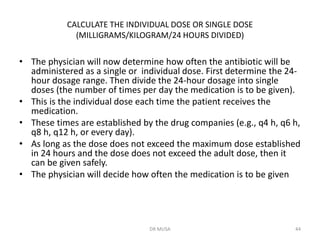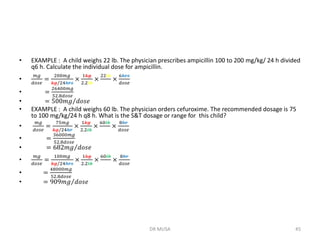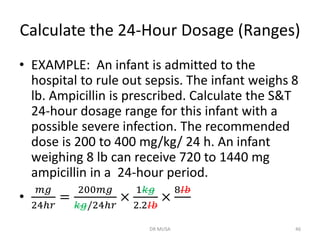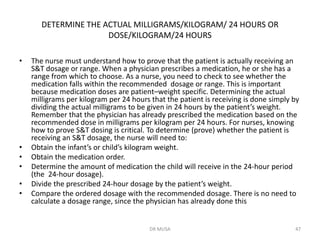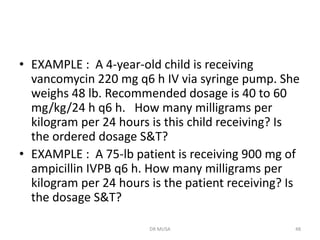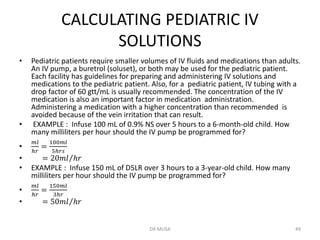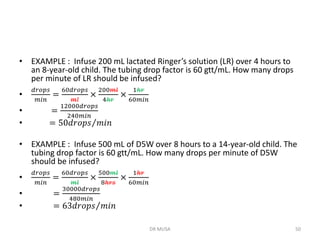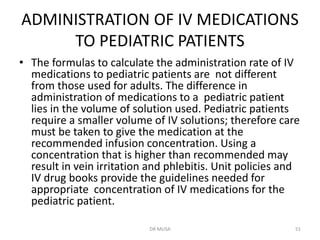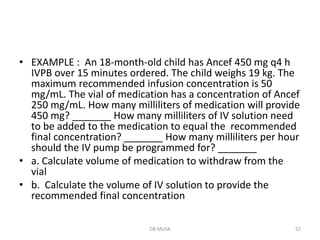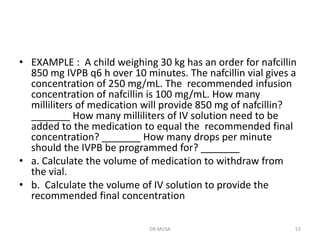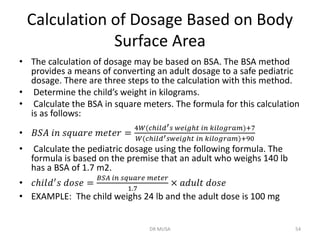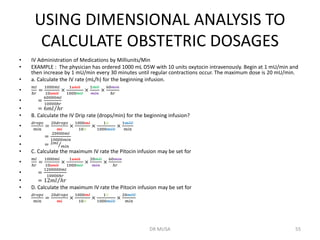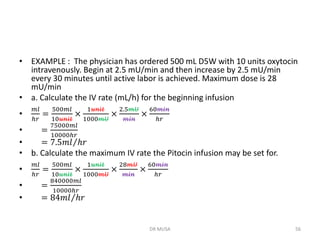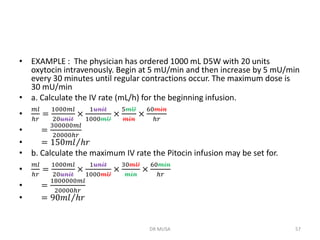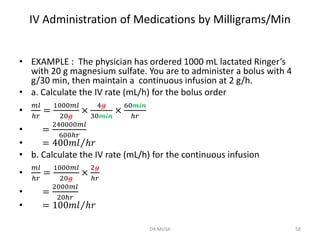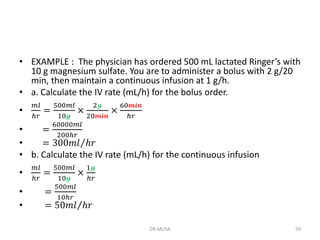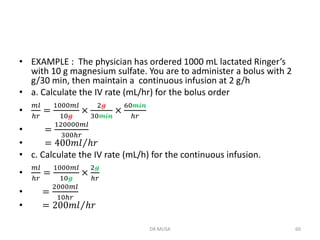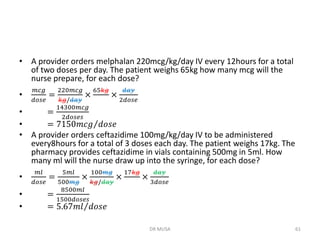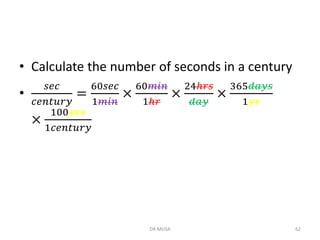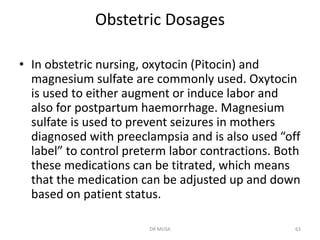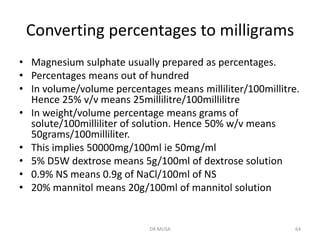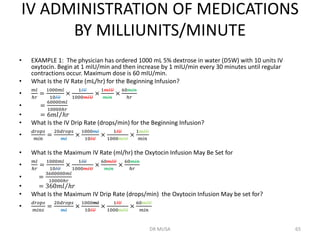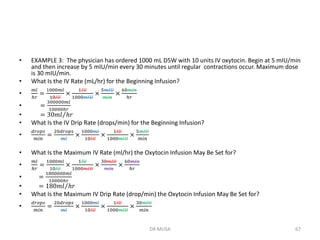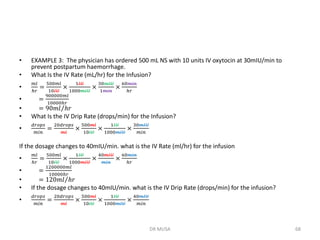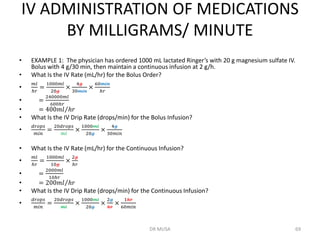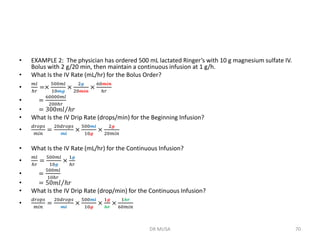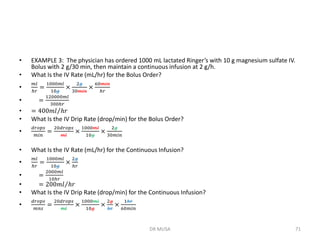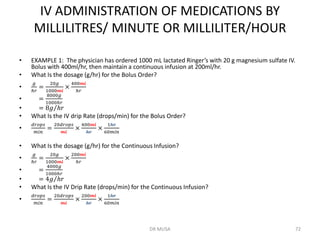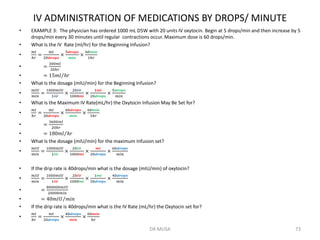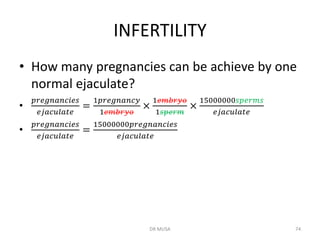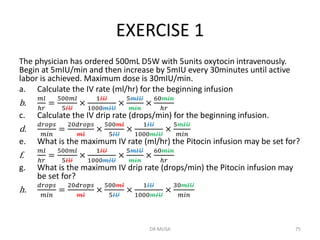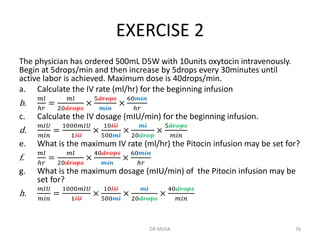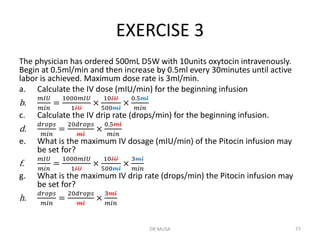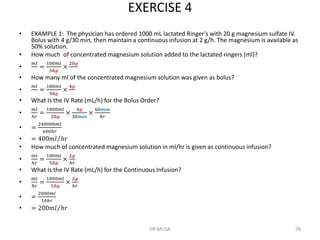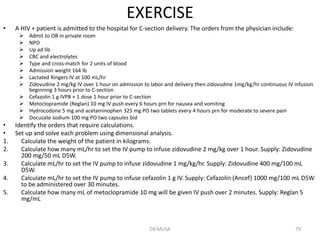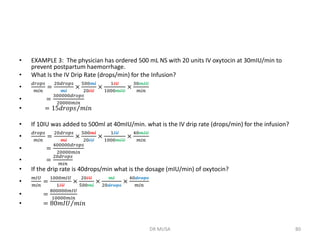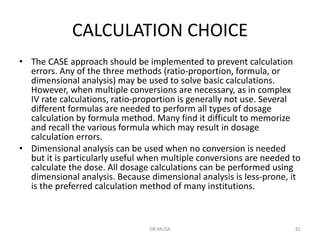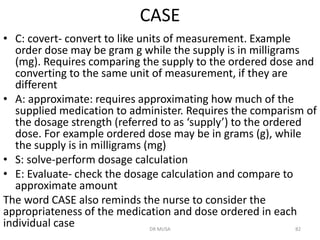1 de 83

### Dimensional analysis-1 [Autosaved].pdf

1. DIMENSIONAL ANALYSIS Dr Musa Marena DR MUSA 1
2. LEARNING OBJECTIVE Solve simple problems by using Dimensional Analysis. Identify some common units of measurement and their abbreviations. Construct unit fractions from equivalences. Convert a quantity expressed with a single unit of measurement to an equivalent quantity with another single unit of measurement. Convert a quantity expressed as a rate to another rate. Solve complex problems using Dimensional Analysis. DR MUSA 2
3. • Using the dimensional analysis format to solve oral dosage problems • Using the dimensional analysis format to solve parenteral dosage problems • Using the dimensional analysis format to solve problems of intravenous flow rate in drops per minute • Using the dimensional analysis format to solve problems involving administration of medications in units per hour • Using the dimensional analysis format to solve problems involving administration of medications in milligrams per minute, micrograms per minute, and micrograms per kilogram per minute • Using the dimensional analysis format to solve pediatric and obstetrical dosage problems DR MUSA 3
4. Background: Dimensional Analysis • Fancy name—simple technique • Used to manipulate units in a calculation • Involves cancellation of unwanted units • Eliminates need to memorize formulas • Also known as the factor-label method or the unit factor method • Only one equation is used
5. Making Conversions Using Dimensional Analysis (cont’d) 5. Identify unwanted or undesired units and cancel, then reduce to lowest terms 6. Must be able to eliminate all labels except for answer label—if not, recheck 7. Perform mathematical process Note: it is key to have the equivalent set up correctly!
6. TERMS • GIVEN QUANTITY: ➢ Values given in the question and includes ❖ Doctor’s order ❖ Dosage to be given ➢ May be use to begin point in answering the question • WANTED QUANTITY: ➢ What you desire ➢ Answer to the problem • UNIT EQUIVALENCY: ➢ Value of equivalencies between two different units ➢ Are not usually given in a question and needs to be memorize • CONVERSION FACTORS: ➢ Unit Equivalent expressed in fraction ➢ Help to cancel out unwanted units from the problem ➢ May be use to begin the solution of the question • UNIT PATH: ➢ Series of conversions necessary to achieve the answer to the problem • UNIT: ➢ A dimension that is given to a number DR MUSA 6
7. • Identify what is been measured • Identify the unit of what is been measured and record it to begin the equation • Write the equal sign after the unit of measure • Identify variable from the either the given quantity or unit equivalent with one of its units similar to the numerator unit of the unit of measure and record it after the equal sign as a conversion factor in such a way that the conversion factor have similar numerator unit as the unit of measure • Add subsequent variables from the given quantity or unit equivalent by such the unwanted units is canceled and the desire units are left • Multiple the numerator values and record with the desire unit left • Multiple the denominator values and record desire unit left • Divide the two values to get desire value record it with the desire units DR MUSA 7
8. TERMS & TERMINOLOGIES • GIVEN QUANTITY: ❖ Quantities/variables give in the problem: includes ❖ Doctor’s order ❖ Dosage to be given ❖ On hand ❖ Some unit equivalents • WANTED QUANTITY: (MEASURE) ❖ What you desire ❖ What you measuring ❖ Answer to the problem • UNIT EQUIVALENCY: ❖ Value of equivalencies between two different units ❖ Are not usually given in the problem ❖ Need to be memorized ❖ Are use in solution as conversion factors • CONVERSION FACTORS: ❖ Unit Equivalent expressed in fraction ❖ Help to cancel out unwanted units from the problem • UNIT PATH: ❖ Series of conversions necessary to achieve the answer to the problem • UNIT: ❖ A dimension that is given to a number • FLOW RATE: volume of fluid administer per unit time • DRIP RATE: number of drops per unit time • CONCENTRATION: weight of solute in a unit volume of solution • DOSAGE: weight of medication per unit time administered DR MUSA 8
9. DROP FACTOR: Is the number of drops through the eye of dropper of a given set that is required to move (infuse) 1ml of the fluid into the drip chamber (i.e. patient). The label on the tubing box/packet will indicate the dropper capacity of the specific tubing used*. The calibration of IV tubing in gtt/ml is known as the drop factor. Common macrodrop factors are 10 gtts/mL, 15gtts/mL, 20gtts/mL and the common microdrop factor are 40gtts/ml, 50gtts/ml and 60gtts/mL. Determine drip capacity by choosing the microdrop chamber or macrodrip chamber. A MICRO OR MINI DRIP SET which delivers 40-60gtts/ml • All medicated infusion to give as accurately as possible and to minimized overdose. tubing is narrower and so produces smaller drops. • children and infants (paediatrics), • infusion sensitive medications where precision in the flow rate is essential (critical care) • If you are infusing any fluid UNDER 60 ML/HR Microdrip tubing (sometimes called minidrip) comes in only one size: 60 gtt/mL. when it is use as the IV infusion set, the Flow rate value is always equal to the drip rate value. DR MUSA 9
10. Mathematical foundation for dimensional analysis • Concept 1: • when a non zero quantity is divided by the same amount the result is 1 • Concept 2: • When a quantity is multiply by 1, the quantity is unchanged DR MUSA 10
11. • There is more than one way to set up a dimensional analysis equation. You can begin with: ➢ the given quantity (desired dose) ➢ A unit equivalent who has a unit similar to the numerator unit of the units of measure(wanted quantity). • There are four ways to set up the equation. No matter which fraction placement you use, the equation is designed for multiplication and cancellation. Three of the following examples start with the given quantity, and one starts with the wanted quantity. One problem will be used to demonstrate each of the four approaches. DR MUSA 11
12. METHODS In both methods identify the dimensions of the wanted Quantity (unit of measure): • SEQUENTIAL: ❖The conversion factors are place in the Unit Path such that the dimensions sequentially cancels those adjacent to them. Begin with either ➢Given quantity ➢A unit equivalence with one of its units similar to the numerator unit of the units of measure (wanted quantity) • RANDOM: ❖The conversion factors are randomly place in the Unit Path such that the dimensions may cancels those away from to them. Begin with either ➢Given Quantity ➢Wanted Quantity DR MUSA 12
13. 1. The order is infuse Pitocin 20U in 500ml of D5W at 100microdrops/min . Calculate the mU/min, ml/hr and mU/hr that the patient will receive. • Measure is dosage • Unit of measure mU/min • Microdrop means calibration of the IV set is 60drops given for every ml. 100microdrop/min means 100drops/min was given using IV set which gives 60drops for every milliliter • mU/min = 1000mU/1U × 20U/500ml × 1ml/60drops × 100drops/min • = 2000000mU/30000min • = 66.67mU/min • Measure is Flow rate • Unit of measure ml/hr • 100micrdrop/min means 100drops/min was given using IV set which gives 60drops for every milliliter • ml/hr = 1ml/60drops × 100drops/min × 60min/hr • = 6000ml/60hr • = 100ml/hr • Measure is dosage • Unit of measure mU/hr • 100micrdrop/min means 100drops/min was given using IV set which gives 60drops for every milliliter • mU/hr = 1000mU/1U × 20U/500ml × 1ml/60drops × 100drops/min × 60min/hr • = 120000000mU/30000hr • = 4000mU/hr DR MUSA 13
14. DR MUSA 14 ml hr 1ml 60dr ops 100d rops min 60min hr 6000 ml 60hr 100 ml hr mU hr 100 0m U 1U 20 U 500 ml 1m l 60 dro ps 10 0d ro ps mi n 60 mi n hr 12 00 00 00 0 m U 3 0 0 0 0 40 00 m U hr
15. Unit of mU/min Microdrops implies IV infusion set use gives 60drops for every 1ml infused 100microdrops/min implies 100drops/min was given using an infusion set that gives 60drops for every 1ml 100ml 6000ml in hr 60hr • Measure is Flow rate • Unit of measure ml/hr • 100micrdrop/min means 100drops/min was given using IV set which gives 60drops for every milliliter 66.67mU 200000m U 100 drop s 1ml 20U 1000m U mU min 30000min min 60drop s 500m l 1U min DR MUSA 15
16. 1. Order: Drug A 20mg for child. Available: Drug A 20mg in 5ml vial. Dilute to 50ml and administer in 2hr. What is the flow rate in drops per minutes? ▪ Medicated infusion, infusion rates less than 60ml/hr, sensitive medication that requires precision in their infusion rate (critical care) or paediatric (infants and children) fluid infusion should always use microdrip IV set with 60drops per ml ▪ Measure is flow rate ▪ Unit of measure drops per minutes drops/min ▪ drops/min = 60drops/ml x 50ml/2hr x 1hr/60min = 3000drops/60min = 50drops/min DR MUSA 16
17. 1. A doctor orders pitocin drip at 45microdrips per minute. The solution contains 20U of Pitocin in 1000ml of D5W. Calculate the units per minute, milliliter per hour and the unit per hour the patient is receiving. • Measure is dosage • Unit of measure is unit per minute ie U/min • Note microdrips means the set calibration is 60drops/ml • U/hr = 20U/1000ml × 1ml/60drops × 45drops/min • = 900U/60000min • = 0.015U/min • • • Measure is Flow rate • Unit of measure ml/hr • 45micrdrop/min means 45drops/min was given using IV set which gives 60drops for every milliliter • mg/hr = 1ml/60drops × 45drops/min × 60mins/hr • = 2700ml/60hr • = 45ml/hr • • Measure is dosage • Unit of measure is unit per hour ie U/hr • U/hr = 20U/1000ml × ml/60drops × 45drops/min × 60min/hr • = 54000U/60000hr • = 0.9U/hr DR MUSA 17
18. 1. The order is infuse Dopamine 400mg in 250ml of D5W at 30microdrops/min . Calculate the mg/min, ml/hr and mg/hr that the patient will receive. • • Measure is dosage • Unit of measure mg/min • Microdrop means calibration of the IV set is 60drops given for every ml. 30microdrop/min means 30drops/min was given using IV set which gives 60drops for every milliliter • mg/min = 400mg/250ml × ml/60drops × 30drops/min • = 12000mg/15000min • = 0.8mg/min • • Measure is flow rate • Microdrop/min implies that the IV set use gives 60drops for every milliliter • Unit of measure ml/hr • ml/hr = 1ml/60drops x 30drops/min x 60min/1hr • = 1800ml/60hr • = 30ml/hr • • Measure is dosage • Unit of measure mg/hr • 30micrdrop/min means 30drops/min was given using IV set which gives 60drops for every milliliter • mg/hr = 400mg/250ml × ml/60drops × 30drops/min × 60min/hr • = 720000mg/15000hr • = 48mg/hr DR MUSA 18
19. EXAMPLE The physician has ordered 500mL D5W with 5units oxytocin intravenously. Begin at 5mU/min and then increase by 5mU every 30minutes until active labor is achieved. Maximum dose is 30mU/min. a. Calculate the IV rate (ml/hr) for the beginning infusion? b. What is the maximum IV drip rate (drops/min) the Pitocin infusion may be set for? DR MUSA 19
20. Sequential Method 1 • Identify the Wanted Quantity and Unit(unit of measure)↔ 𝑑𝑟𝑜𝑝𝑠 𝑚𝑖𝑛 • Identify the Unit Equivalencies and Conversion Factors write them as Unit equivalencies • 5𝑈 = 500𝑚𝑙; 1U = 1000mU; 20drops = ml; 1hr = 60min; 30mU = min • Create a unit paths ➢ Write dimensions of wanted quantity(unit of measure) as Conversion factor to begin the equation: 𝑑𝑟𝑜𝑝𝑠 𝑚𝑖𝑛 ➢ Write an equal sign: 𝑑𝑟𝑜𝑝𝑠 𝑚𝑖𝑛 = ➢ Begin with a Unit Equivalent that has drops as one of its dimension : 𝑑𝑟𝑜𝑝𝑠 𝑚𝑖𝑛 = 𝑑𝑟𝑜𝑝𝑠 ❖ unit equivalent with drops as its one of its dimension: 20𝑑𝑟𝑜𝑝𝑠 = 𝑚𝑙 ➢ Write the Unit Equivalent as conversion factor with it numerator unit similar to that of the wanted Quantity: ➢ 𝑑𝑟𝑜𝑝𝑠 𝑚𝑖𝑛 = 20𝑑𝑟𝑜𝑝𝑠 𝑚𝑙 ➢ Add the subsequent conversion factors so that their numerator dimension is similar to the denominator unit of the preceding conversion factor. This will allow Unwanted quantity to cancel each other leaving only the Wanted dimensions. ➢ 𝑑𝑟𝑜𝑝𝑠 𝑚𝑖𝑛 = 20𝑑𝑟𝑜𝑝𝑠 𝑚𝑙 ×⇗ 𝑚𝑙 ➢ This process continue until all the Unwanted units are cancelled out leaving only the desire(Wanted dimension ie the Dimensions of Wanted Quantity) ➢ Multiple the numerators: 𝑑𝑟𝑜𝑝𝑠 𝑚𝑖𝑛 = 20𝑑𝑟𝑜𝑝𝑠 𝑚𝑙 × 500𝑚𝑙 5𝑈 × 1𝑈 1000𝑚𝐼𝑈 × 30𝑚𝐼𝑈 𝑚𝑖𝑛 = 300000𝑑𝑟𝑜𝑝𝑠 ➢ Multiple the denominators: : 𝑑𝑟𝑜𝑝𝑠 𝑚𝑖𝑛 = 20𝑑𝑟𝑜𝑝𝑠 𝑚𝑙 × 500𝑚𝑙 5𝑈 × 1𝑈 1000𝑚𝐼𝑈 × 30𝑚𝐼𝑈 𝑚𝑖𝑛 = 300000𝑑𝑟𝑜𝑝𝑠 5000𝑚𝑖𝑛 ➢ Divide the results: : 𝑑𝑟𝑜𝑝𝑠 𝑚𝑖𝑛 = 20𝑑𝑟𝑜𝑝𝑠 𝑚𝑙 × 500𝑚𝑙 5𝑈 × 1𝑈 1000𝑚𝐼𝑈 × 30𝑚𝐼𝑈 𝑚𝑖𝑛 = 300000𝑑𝑟𝑜𝑝𝑠 5000𝑚𝑖𝑛 = 60 𝑑𝑟𝑜𝑝𝑠 𝑚𝑖𝑛 DR MUSA 20
21. SEQUENTIAL METHOD 1a a. Wanted Quantity↔ 𝐹𝑙𝑜𝑤 𝑅𝑎𝑡𝑒 ➢ Dimension of wanted Quantity(unit of measure)↔ 𝑚𝑙 ℎ𝑟 b. Unit Equivalencies (conversion Factors) ➢ 500𝑚𝑙 = 5𝑢𝑛𝑖𝑡 ➢ 1𝑢𝑛𝑖𝑡 = 1000𝑚𝐼𝑈 ➢ 5𝑚𝐼𝑈 = 𝑚𝑖𝑛 ➢ 60𝑚𝑖𝑛 = 1ℎ𝑟 c. Unit Path Way ➢ 𝑚𝑙 ℎ𝑟 = ➢ 𝑚𝑙 ℎ𝑟 = 500𝑚𝑙 5𝑢𝑛𝑖𝑡 ➢ 𝑚𝑙 ℎ𝑟 = 500𝑚𝑙 5𝑢𝑛𝑖𝑡 × 1𝑢𝑛𝑖𝑡 1000𝑚𝐼𝑈 ➢ 𝑚𝑙 ℎ𝑟 = 500𝑚𝑙 5𝑢𝑛𝑖𝑡 × 1𝑢𝑛𝑖𝑡 1000𝑚𝐼𝑈 × 5𝑚𝐼𝑈 𝑚𝑖𝑛 ➢ 𝑚𝑙 ℎ𝑟 = 500𝑚𝑙 5𝑢𝑛𝑖𝑡 × 1𝑢𝑛𝑖𝑡 1000𝑚𝐼𝑈 × 5𝑚𝐼𝑈 𝑚𝑖𝑛 × 60𝑚𝑖𝑛 ℎ𝑟 ➢ 𝑚𝑙 ℎ𝑟 = 500𝑚𝑙 5unit × 1𝑢𝑛𝑖𝑡 1000𝑚𝐼𝑈 × 5𝑚𝐼𝑈 𝑚𝑖𝑛 × 60𝑚𝑖𝑛 1ℎ𝑟 = 150000𝑚𝑙 5000ℎ𝑟 ➢ 𝑚𝑙 ℎ𝑟 = 500𝑚𝑙 5𝑢𝑛𝑖𝑡 × 1𝑢𝑛𝑖𝑡 1000𝑚𝐼𝑈 × 5𝑚𝐼𝑈 𝑚𝑖𝑛 × 60𝑚𝑖𝑛 ℎ𝑟 = 150000𝑚𝑙 5000ℎ𝑟 = 30𝑚𝑙 ℎ𝑟 DR MUSA 21
22. SEQUENTIAL METHOD 1b a. Wanted Quantity↔ 𝐹𝑙𝑜𝑤 𝑅𝑎𝑡𝑒 ➢ Dimension of wanted Quantity(unit of measure)↔ 𝑚𝑙 ℎ𝑟 b. Unit Equivalencies (conversion Factors) ➢ 500𝑚𝑙 = 5𝑢𝑛𝑖𝑡 ➢ 1𝑢𝑛𝑖𝑡 = 1000𝑚𝐼𝑈 ➢ 5𝑚𝐼𝑈 = 𝑚𝑖𝑛 ➢ 60𝑚𝑖𝑛 = 1ℎ𝑟 c. Unit Path Way ➢ = 𝑚𝑙 ℎ𝑟 ➢ 500𝑚𝑙 5𝑢𝑛𝑖𝑡 = 𝑚𝑙 ℎ𝑟 ➢ 500𝑚𝑙 5𝑢𝑛𝑖𝑡 × 1𝑢𝑛𝑖𝑡 1000𝑚𝐼𝑈 = 𝑚𝑙 ℎ𝑟 ➢ 500𝑚𝑙 5𝑢𝑛𝑖𝑡 × 1𝑢𝑛𝑖𝑡 1000𝑚𝐼𝑈 × 5𝑚𝐼𝑈 𝑚𝑖𝑛 = 𝑚𝑙 ℎ𝑟 ➢ 500𝑚𝑙 5𝑢𝑛𝑖𝑡 × 1𝑢𝑛𝑖𝑡 1000𝑚𝐼𝑈 × 5𝑚𝐼𝑈 𝑚𝑖𝑛 × 60𝑚𝑖𝑛 ℎ𝑟 = 𝑚𝑙 ℎ𝑟 ➢ 500𝑚𝑙 5unit × 1𝑢𝑛𝑖𝑡 1000𝑚𝐼𝑈 × 5𝑚𝐼𝑈 𝑚𝑖𝑛 × 60𝑚𝑖𝑛 1ℎ𝑟 = 150000𝑚𝑙 5000ℎ𝑟 ➢ 500𝑚𝑙 5𝑢𝑛𝑖𝑡 × 1𝑢𝑛𝑖𝑡 1000𝑚𝐼𝑈 × 5𝑚𝐼𝑈 𝑚𝑖𝑛 × 60𝑚𝑖𝑛 ℎ𝑟 = 150000𝑚𝑙 5000ℎ𝑟 = 30𝑚𝑙 ℎ𝑟 DR MUSA 22
23. SEQUENTIAL METHOD 2 • Identify the Wanted Quantity and Unit (unit of measure)↔ 𝑑𝑟𝑜𝑝𝑠 𝑚𝑖𝑛 • Identify the Unit Equivalencies and Conversion Factors write them as Unit equivalencies ➢ 5𝑈 = 500𝑚𝑙; 1U = 1000mU; 20drops = ml; 1hr = 60min; 30mIU = min • Identify the Given Quantity with Unit↔ 30𝑚𝑈 𝑚𝑖𝑛 • Create a unit paths ➢ Write dimensions of wanted quantity(unit of measure) as Conversion factor to begin the equation: 𝑑𝑟𝑜𝑝𝑠 𝑚𝑖𝑛 ➢ Write an equal sign: 𝑑𝑟𝑜𝑝𝑠 𝑚𝑖𝑛 = ➢ Write the given Quantity as Conversion Factor: 𝑑𝑟𝑜𝑝𝑠 𝑚𝑖𝑛 = 30𝑚𝑈 𝑚𝑖𝑛 ➢ Add the rest of Unit Equivalencies as multiples of Conversion factors so that one of the Unwanted Unit of the preceding variable is cancelled out by an Unwanted unit of the succeeding variable: ➢ 𝑑𝑟𝑜𝑝𝑠 𝑚𝑖𝑛 = 30𝑚𝑈 𝑚𝑖𝑛 × 1𝑈 1000𝑚𝑈 × 500𝑚𝑙 5𝑈 × 20𝑑𝑟𝑜𝑝𝑠 𝑚𝑙 ➢ This process continue until all the Unwanted units are cancelled out leaving only the desire(Wanted dimension ie the Dimensions of Wanted Quantity) ➢ Multiple the numerators: 𝑑𝑟𝑜𝑝𝑠 𝑚𝑖𝑛 = 30𝑚𝐼𝑈 𝑚𝑖𝑛 × 1𝑈 1000𝑚𝐼𝑈 × 500𝑚𝑙 5𝑈 × 20𝑑𝑟𝑜𝑝𝑠 𝑚𝑙 = 300000𝑑𝑟𝑜𝑝𝑠 ➢ Multiple the denominators: 𝑑𝑟𝑜𝑝𝑠 𝑚𝑖𝑛 = 30𝑚𝐼𝑈 𝑚𝑖𝑛 × 1𝑈 1000𝑚𝐼𝑈 × 500𝑚𝑙 5𝑈 × 20𝑑𝑟𝑜𝑝𝑠 𝑚𝑙 = 300000𝑑𝑟𝑜𝑝𝑠 5000𝑚𝑖𝑛 ➢ Divide the results: 𝑑𝑟𝑜𝑝𝑠 𝑚𝑖𝑛 = 30𝑚𝐼𝑈 𝑚𝑖𝑛 × 1𝑈 1000𝑚𝐼𝑈 × 500𝑚𝑙 5𝑈 × 20𝑑𝑟𝑜𝑝𝑠 𝑚𝑙 = 300000𝑑𝑟𝑜𝑝𝑠 5000𝑚𝑖𝑛 = 60 𝑑𝑟𝑜𝑝𝑠 𝑚𝑖𝑛 DR MUSA 23
24. SEQUENTIAL METHOD 2a • Wanted quantity↔ 𝐹𝑙𝑜𝑤 𝑅𝑎𝑡𝑒 ➢ Dimensions of Wanted Quantity(unit of measure)↔ 𝑚𝑙 ℎ𝑟 • Unit Equivalencies(Conversion Factors) ➢ 1𝑢𝑛𝑖𝑡 = 1000𝑚𝐼𝑈 ➢ 500𝑚𝑙 = 5𝑢𝑛𝑖𝑡 ➢ 60𝑚𝑖𝑛 = 1ℎ𝑟 ➢ 5𝑚𝐼𝑈 = 𝑚𝑖𝑛 • Given Quantity↔ 5𝑚𝐼𝑈 𝑚𝑖𝑛 • Unit Path Way ➢ = 𝑚𝑙 ℎ𝑟 ➢ 5𝑚𝐼𝑈 𝑚𝑖𝑛 = 𝑚𝑙 ℎ𝑟 ➢ 5𝑚𝐼𝑈 𝑚𝑖𝑛 × 1𝑢𝑖𝑛𝑡 1000𝑚𝐼𝑈 = 𝑚𝑙 ℎ𝑟 ➢ 5𝑚𝐼𝑈 𝑚𝑖𝑛 × 1𝑢𝑛𝑖𝑡 1000𝑚𝐼𝑈 × 500𝑚𝑙 5𝑢𝑛𝑖𝑡 = 𝑚𝑙 ℎ𝑟 ➢ 5𝑚𝐼𝑈 𝑚𝑖𝑛 × 1𝑢𝑛𝑖𝑡 1000𝑚𝐼𝑈 × 500𝑚𝑙 5𝑢𝑛𝑖𝑡 × 60𝑚𝑖𝑛 ℎ𝑟 = 𝑚𝑙 ℎ𝑟 ➢ 5𝑚𝐼𝑈 𝑚𝑖𝑛 × 1𝑢𝑛𝑖𝑡 1000𝑚𝐼𝑈 × 500𝑚𝑙 5𝑢𝑛𝑖𝑡 × 60𝑚𝑖𝑛 ℎ𝑟 = 150000𝑚𝑙 5000ℎ𝑟 ➢ 5𝑚𝐼𝑈 𝑚𝑖𝑛 × 1𝑢𝑛𝑖𝑡 1000𝑚𝐼𝑈 × 500𝑚𝑙 5𝑢𝑛𝑖𝑡 × 60𝑚𝑖𝑛 ℎ𝑟 = 150000𝑚𝑙 5000ℎ𝑟 = 30𝑚𝑙 ℎ𝑟 DR MUSA 24
25. SEQUENTIAL METHODS 2b • Wanted quantity↔ 𝐹𝑙𝑜𝑤 𝑅𝑎𝑡𝑒 ➢ Dimensions of Wanted Quantity(unit of measure)↔ 𝑚𝑙 ℎ𝑟 • Unit Equivalencies(Conversion Factors) ➢ 1𝑢𝑛𝑖𝑡 = 1000𝑚𝐼𝑈 ➢ 500𝑚𝑙 = 5𝑢𝑛𝑖𝑡 ➢ 60𝑚𝑖𝑛 = 1ℎ𝑟 ➢ 5𝑚𝐼𝑈 = 𝑚𝑖𝑛 • Given Quantity↔ 5𝑚𝐼𝑈 𝑚𝑖𝑛 • Unit Path Way ➢ 𝑚𝑙 ℎ𝑟 = ➢ 𝑚𝑙 ℎ𝑟 = 5𝑚𝐼𝑈 𝑚𝑖𝑛 ➢ 𝑚𝑙 ℎ𝑟 = 5𝑚𝐼𝑈 𝑚𝑖𝑛 × 1𝑢𝑖𝑛𝑡 1000𝑚𝐼𝑈 ➢ 𝑚𝑙 ℎ𝑟 = 5𝑚𝐼𝑈 𝑚𝑖𝑛 × 1𝑢𝑛𝑖𝑡 1000𝑚𝐼𝑈 × 500𝑚𝑙 5𝑢𝑛𝑖𝑡 ➢ 𝑚𝑙 ℎ𝑟 = 5𝑚𝐼𝑈 𝑚𝑖𝑛 × 1𝑢𝑛𝑖𝑡 1000𝑚𝐼𝑈 × 500𝑚𝑙 5𝑢𝑛𝑖𝑡 × 60𝑚𝑖𝑛 ℎ𝑟 ➢ 𝑚𝑙 ℎ𝑟 = 5𝑚𝐼𝑈 𝑚𝑖𝑛 × 1𝑢𝑛𝑖𝑡 1000𝑚𝐼𝑈 × 500𝑚𝑙 5𝑢𝑛𝑖𝑡 × 60𝑚𝑖𝑛 ℎ𝑟 = 150000𝑚𝑙 5000ℎ𝑟 ➢ 𝑚𝑙 ℎ𝑟 = 5𝑚𝐼𝑈 𝑚𝑖𝑛 × 1𝑢𝑛𝑖𝑡 1000𝑚𝐼𝑈 × 500𝑚𝑙 5𝑢𝑛𝑖𝑡 × 60𝑚𝑖𝑛 ℎ𝑟 = 150000𝑚𝑙 5000ℎ𝑟 = 30𝑚𝑙 ℎ𝑟 DR MUSA 25
26. RANDOM METHOD 1 • Identify the Wanted Quantity and Unit(unit of measure)↔ 𝑑𝑟𝑜𝑝𝑠 𝑚𝑖𝑛 • Identify the Unit Equivalencies and Conversion Factors write them as Unit equivalents ➢ 5𝑈 = 500𝑚𝑙; 1U = 1000mU; 20drops = ml; 1hr = 60min; 30mIU = min • Identify the Given Quantity with Unit↔ 30𝑚𝑈 𝑚𝑖𝑛 • Create a unit paths ➢ Write dimensions of wanted quantity (unit of measure)as Conversion factor to begin the equation: 𝑑𝑟𝑜𝑝𝑠 𝑚𝑖𝑛 ➢ Write an equal sign: 𝑑𝑟𝑜𝑝𝑠 𝑚𝑖𝑛 = ➢ Write the given Quantity as Conversion Factor: 𝑑𝑟𝑜𝑝𝑠 𝑚𝑖𝑛 = 30𝑚𝑈 𝑚𝑖𝑛 ➢ Add the rest of Unit Equivalencies as multiples of Conversion factors without order so that one of the Unwanted Unit are cancelled and only the desire or wanted dimensions are left: ➢ 𝑑𝑟𝑜𝑝𝑠 𝑚𝑖𝑛 = 30𝑚𝐼𝑈 𝑚𝑖𝑛 × 500𝑚𝑙 5𝑈 × 1𝑈 1000𝑚𝐼𝑈 × 20𝑑𝑟𝑜𝑝𝑠 𝑚𝑙 ➢ Multiple the numerators: 𝑑𝑟𝑜𝑝𝑠 𝑚𝑖𝑛 = 30𝑚𝐼𝑈 𝑚𝑖𝑛 × 500𝑚𝑙 5𝑈 × 1𝑈 1000𝑚𝐼𝑈 × 20𝑑𝑟𝑜𝑝𝑠 𝑚𝑙 = 300000𝑑𝑟𝑜𝑝𝑠 ➢ Multiple the denominators: 𝑑𝑟𝑜𝑝𝑠 𝑚𝑖𝑛 = 30𝑚𝐼𝑈 𝑚𝑖𝑛 × 500𝑚𝑙 5𝑈 × 1𝑈 1000𝑚𝐼𝑈 × 20𝑑𝑟𝑜𝑝𝑠 𝑚𝑙 = 300000𝑑𝑟𝑜𝑝𝑠 5000𝑚𝑖𝑛 ➢ Divide the results: 𝑑𝑟𝑜𝑝𝑠 𝑚𝑖𝑛 = 30𝑚𝐼𝑈 𝑚𝑖𝑛 × 500𝑚𝑙 5𝑈 × 1𝑈 1000𝑚𝐼𝑈 × 20𝑑𝑟𝑜𝑝𝑠 𝑚𝑙 = 300000𝑑𝑟𝑜𝑝𝑠 5000𝑚𝑖𝑛 = 60 𝑑𝑟𝑜𝑝𝑠 𝑚𝑙 DR MUSA 26
27. RANDOM METHOD 2 • Identify the Wanted Quantity and Unit(unit of measure)↔ 𝑑𝑟𝑜𝑝𝑠 𝑚𝑖𝑛 • Identify the Unit Equivalencies and Conversion Factors write them as Unit equivalents ➢ 5𝑈 = 500𝑚𝑙; 1U = 1000mU; 20drops = ml; 1hr = 60min; 30mIU = min • Identify unit equivalent that have dimension similar to the numerator of the wanted Quantity with Unit↔ 20𝑑𝑟𝑜𝑝𝑠 = 𝑚𝑙; 20𝑑𝑟𝑜𝑝𝑠 𝑚𝑙 • Create a unit paths ➢ Write dimensions of wanted quantity (unit of measure) as Conversion factor to begin the equation: 𝑑𝑟𝑜𝑝𝑠 𝑚𝑖𝑛 ➢ Write an equal sign: 𝑑𝑟𝑜𝑝𝑠 𝑚𝑖𝑛 = ➢ Write the given Quantity as Conversion Factor: 𝑑𝑟𝑜𝑝𝑠 𝑚𝑖𝑛 = 20𝑑𝑟𝑜𝑝𝑠 𝑚𝑙 ➢ Add the rest of Unit Equivalent as multiples of Conversion factors without order so that one of the Unwanted Unit are cancelled and only the desire or wanted dimensions are left: ➢ 𝑑𝑟𝑜𝑝𝑠 𝑚𝑖𝑛 = 20𝑑𝑟𝑜𝑝𝑠 𝑚𝑙 × 30𝑚𝐼𝑈 𝑚𝑖𝑛 × 500𝑚𝑙 5𝑈 × 1𝑈 1000𝑚𝐼𝑈 ➢ Multiple the numerators: 𝑑𝑟𝑜𝑝𝑠 𝑚𝑖𝑛 = 20𝑑𝑟𝑜𝑝𝑠 𝑚𝑙 × 30𝑚𝐼𝑈 𝑚𝑖𝑛 × 500𝑚𝑙 5𝑈 × 1𝑈 1000𝑚𝐼𝑈 = 300000𝑑𝑟𝑜𝑝𝑠 ➢ Multiple the denominators: 𝑑𝑟𝑜𝑝𝑠 𝑚𝑖𝑛 = 20𝑑𝑟𝑜𝑝𝑠 𝑚𝑙 × 30𝑚𝐼𝑈 𝑚𝑖𝑛 × 500𝑚𝑙 5𝑈 × 1𝑈 1000𝑚𝐼𝑈 = 300000𝑑𝑟𝑜𝑝𝑠 5000𝑚𝑖𝑛 ➢ Divide the results: 𝑑𝑟𝑜𝑝𝑠 𝑚𝑖𝑛 = 20𝑑𝑟𝑜𝑝𝑠 𝑚𝑙 × 30𝑚𝐼𝑈 𝑚𝑖𝑛 × 500𝑚𝑙 5𝑈 × 1𝑈 1000𝑚𝐼𝑈 = 300000𝑑𝑟𝑜𝑝𝑠 5000𝑚𝑖𝑛 = 60 𝑑𝑟𝑜𝑝𝑠 𝑚𝑙 DR MUSA 27
28. RANDOM METHOD 1a • Wanted quantity↔ 𝐹𝑙𝑜𝑤 𝑅𝑎𝑡𝑒 ➢ Dimensions of Wanted Quantity(unit of measure)↔ 𝑚𝑙 ℎ𝑟 • Unit Equivalencies(Conversion Factors) ➢ 1𝑢𝑛𝑖𝑡 = 1000𝑚𝐼𝑈 ➢ 500𝑚𝑙 = 5𝑢𝑛𝑖𝑡 ➢ 60𝑚𝑖𝑛 = 1ℎ𝑟 ➢ 5𝑚𝐼𝑈 = 𝑚𝑖𝑛 • Given Quantity↔ 5𝑚𝐼𝑈 𝑚𝑖𝑛 • Unit Path Way ➢ = 𝑚𝑙 ℎ𝑟 ➢ 5𝑚𝐼𝑈 𝑚𝑖𝑛 = 𝑚𝑙 ℎ𝑟 ➢ 5𝑚𝐼𝑈 𝑚𝑖𝑛 × 500𝑚𝑙 5𝑢𝑛𝑖𝑡 = 𝑚𝑙 ℎ𝑟 ➢ 5𝑚𝐼𝑈 𝑚𝑖𝑛 × 500𝑚𝑙 5𝑢𝑛𝑖𝑡 × 1𝑢𝑛𝑖𝑡 1000𝑚𝐼𝑈 = 𝑚𝑙 ℎ𝑟 ➢ 5𝑚𝐼𝑈 𝑚𝑖𝑛 × 500𝑚𝑙 5𝑢𝑛𝑖𝑡 × 1𝑢𝑛𝑖𝑡 1000𝑚𝐼𝑈 × 60𝑚𝑖𝑛 ℎ𝑟 = 𝑚𝑙 ℎ𝑟 ➢ 5𝑚𝐼𝑈 𝑚𝑖𝑛 × 500𝑚𝑙 5𝑢𝑛𝑖𝑡 × 1𝑢𝑛𝑖𝑡 1000𝑚𝐼𝑈 × 60𝑚𝑖𝑛 ℎ𝑟 = 150000𝑚𝑙 5000ℎ𝑟 = 30𝑚𝑙 ℎ𝑟 DR MUSA 28
29. RANDOM METHOD 1b • Wanted quantity↔ 𝐹𝑙𝑜𝑤 𝑅𝑎𝑡𝑒 ➢ Dimensions of Wanted Quantity(unit of measure)↔ 𝑚𝑙 ℎ𝑟 • Unit Equivalents(Conversion Factors) ➢ 1𝑢𝑛𝑖𝑡 = 1000𝑚𝐼𝑈 ➢ 500𝑚𝑙 = 5𝑢𝑛𝑖𝑡 ➢ 60𝑚𝑖𝑛 = 1ℎ𝑟 ➢ 5𝑚𝐼𝑈 = 𝑚𝑖𝑛 • Given Quantity↔ 5𝑚𝐼𝑈 𝑚𝑖𝑛 • Unit Path Way ➢ 𝑚𝑙 ℎ𝑟 = ➢ 𝑚𝑙 ℎ𝑟 = 5𝑚𝐼𝑈 𝑚𝑖𝑛 ➢ 𝑚𝑙 ℎ𝑟 = 5𝑚𝐼𝑈 𝑚𝑖𝑛 × 500𝑚𝑙 5𝑢𝑛𝑖𝑡 ➢ 𝑚𝑙 ℎ𝑟 = 5𝑚𝐼𝑈 𝑚𝑖𝑛 × 500𝑚𝑙 5𝑢𝑛𝑖𝑡 × 1𝑢𝑛𝑖𝑡 1000𝑚𝐼𝑈 ➢ 𝑚𝑙 ℎ𝑟 = 5𝑚𝐼𝑈 𝑚𝑖𝑛 × 500𝑚𝑙 5𝑢𝑛𝑖𝑡 × 1𝑢𝑛𝑖𝑡 1000𝑚𝐼𝑈 × 60𝑚𝑖𝑛 ℎ𝑟 ➢ 𝑚𝑙 ℎ𝑟 = 5𝑚𝐼𝑈 𝑚𝑖𝑛 × 500𝑚𝑙 5𝑢𝑛𝑖𝑡 × 1𝑢𝑛𝑖𝑡 1000𝑚𝐼𝑈 × 60𝑚𝑖𝑛 ℎ𝑟 = 150000𝑚𝑙 5000ℎ𝑟 = 30𝑚𝑙 ℎ𝑟 DR MUSA 29
30. RANDOM METHOD 2a • Wanted quantity↔ 𝐹𝑙𝑜𝑤 𝑅𝑎𝑡𝑒 ➢ Dimensions of Wanted Quantity(unit of measure)↔ 𝑚𝑙 ℎ𝑟 • Unit Equivalencies(Conversion Factors) ➢ 1𝑢𝑛𝑖𝑡 = 1000𝑚𝐼𝑈 ➢ 500𝑚𝑙 = 5𝑢𝑛𝑖𝑡 ➢ 60𝑚𝑖𝑛 = 1ℎ𝑟 ➢ 5𝑚𝐼𝑈 = 𝑚𝑖𝑛 • Unit equivalent with dimensions similar to numerator of the wanted Quantity↔ 500𝑚𝑙 = 5𝑢𝑛𝑖𝑡: 500𝑚𝑙 5𝑢𝑛𝑖𝑡 • Unit Path Way ➢ = 𝑚𝑙 ℎ𝑟 ➢ 500𝑚𝑙 5𝑢𝑛𝑖𝑡 = 𝑚𝑙 ℎ𝑟 ➢ 500𝑚𝑙 5𝑢𝑛𝑖𝑡 × 5𝑚𝐼𝑈 𝑚𝑖𝑛 = 𝑚𝑙 ℎ𝑟 ➢ 500𝑚𝑙 5𝑢𝑛𝑖𝑡 × 5𝑚𝐼𝑈 𝑚𝑖𝑛 × 1𝑢𝑛𝑖𝑡 1000𝑚𝐼𝑈 = 𝑚𝑙 ℎ𝑟 ➢ 500𝑚𝑙 5𝑢𝑛𝑖𝑡 × 5𝑚𝐼𝑈 𝑚𝑖𝑛 × 1𝑢𝑛𝑖𝑡 1000𝑚𝐼𝑈 × 60𝑚𝑖𝑛 ℎ𝑟 = 𝑚𝑙 ℎ𝑟 ➢ 500𝑚𝑙 5𝑢𝑛𝑖𝑡 × 5𝑚𝐼𝑈 min × 1𝑢𝑛𝑖𝑡 1000𝑚𝐼𝑈 × 60𝑚𝑖𝑛 ℎ𝑟 = 150000𝑚𝑙 5000ℎ𝑟 = 30𝑚𝑙 ℎ𝑟 DR MUSA 30
31. RANDOM METHOD 2b • Wanted quantity↔ 𝐹𝑙𝑜𝑤 𝑅𝑎𝑡𝑒 ➢ Dimensions of Wanted Quantity(unit of measure)↔ 𝑚𝑙 ℎ𝑟 • Unit Equivalencies(Conversion Factors) ➢ 1𝑢𝑛𝑖𝑡 = 1000𝑚𝐼𝑈 ➢ 500𝑚𝑙 = 5𝑢𝑛𝑖𝑡 ➢ 60𝑚𝑖𝑛 = 1ℎ𝑟 ➢ 5𝑚𝐼𝑈 = 𝑚𝑖𝑛 • Unit equivalent with dimensions similar to numerator of the wanted Quantity↔ 500𝑚𝑙 = 5𝑢𝑛𝑖𝑡: 𝑖𝑒 500𝑚𝑙 5𝑢𝑛𝑖𝑡 • Unit Path Way ➢ 𝑚𝑙 ℎ𝑟 = ➢ 𝑚𝑙 ℎ𝑟 = 500𝑚𝑙 5𝑢𝑛𝑖𝑡 ➢ 𝑚𝑙 ℎ𝑟 = 500𝑚𝑙 5𝑢𝑛𝑖𝑡 × 5𝑚𝐼𝑈 𝑚𝑖𝑛 ➢ 𝑚𝑙 ℎ𝑟 = 500𝑚𝑙 5𝑢𝑛𝑖𝑡 × 5𝑚𝐼𝑈 𝑚𝑖𝑛 × 1𝑢𝑛𝑖𝑡 1000𝑚𝐼𝑈 ➢ 𝑚𝑙 ℎ𝑟 = 500𝑚𝑙 5𝑢𝑛𝑖𝑡 × 5𝑚𝐼𝑈 𝑚𝑖𝑛 × 1𝑢𝑛𝑖𝑡 1000𝑚𝐼𝑈 × 60𝑚𝑖𝑛 ℎ𝑟 ➢ 𝑚𝑙 ℎ𝑟 = 500𝑚𝑙 5𝑢𝑛𝑖𝑡 × 5𝑚𝐼𝑈 min × 1𝑢𝑛𝑖𝑡 1000𝑚𝐼𝑈 × 60𝑚𝑖𝑛 ℎ𝑟 = 150000𝑚𝑙 5000ℎ𝑟 = 30𝑚𝑙 ℎ𝑟 DR MUSA 31
32. USING DIMENSIONAL ANALYSIS TO CALCULATE IV FLOW RATES • Dimensional analysis is another format for setting up problems to calculate drug dosages. The advantage of dimensional analysis is that only one equation is needed. This is true even if the information supplied indicates a need to convert to like units before setting up the proportion to perform the actual calculation of the amount of medication to be given to the patient. • The dimensional analysis method can also be used to calculate intravenous (IV) flow rates. The following formulas demonstrate how to calculate drops per minute (gtt/min) and milliliters per hour (mL/h). DR MUSA 32
33. • EXAMPLE 1: The physician orders gentamicin 80 mg in 100 mL D5W to be infused over 1 hour. The tubing drop factor is 60 gtt/mL. How many drops per minute should be given to infuse the gentamicin over 1 hour • 𝑑𝑟𝑜𝑝𝑠 𝑚𝑖𝑛 = 60𝑑𝑟𝑜𝑝𝑠 𝑚𝑙 × 100𝑚𝑙 1ℎ𝑟 × 1ℎ𝑟 60𝑚𝑖𝑛 • = 6000𝑑𝑟𝑜𝑝𝑠 60𝑚𝑖𝑛 • = Τ 100𝑑𝑟𝑜𝑝𝑠 𝑚𝑖𝑛 • EXAMPLE 2: The physician orders cefuroxime 1 g in 50 mL of normal saline solution (NS) to be infused over 30 minutes. The tubing drop factor is 60 gtt/mL. How many drops per minute should be given to infuse the total amount of cefuroxime over 30 minutes? • 𝑑𝑟𝑜𝑝𝑠 𝑚𝑖𝑛 = 60𝑑𝑟𝑜𝑝𝑠 𝑚𝑙 × 50𝑚𝑙 30𝑚𝑖𝑛 • = 3000𝑑𝑟𝑜𝑝𝑠 30𝑚𝑖𝑛 • = Τ 100𝑑𝑟𝑜𝑝𝑠 𝑚𝑖𝑛 DR MUSA 33
34. • EXAMPLE 3 : Hespan 500 mL is ordered to be infused over 3 hours. The drop factor is 15 gtt/mL. How many drops per minute should be given to infuse the total amount of Hespan over 3 hours? • 𝑑𝑟𝑜𝑝𝑠 𝑚𝑖𝑛 = 15𝑑𝑟𝑜𝑝𝑠 𝑚𝑙 × 500𝑚𝑙 3ℎ𝑟 × 1ℎ𝑟 60𝑚𝑖𝑛 • = 7500𝑑𝑟𝑜𝑝𝑠 180𝑚𝑖𝑛 • = Τ 42𝑑𝑟𝑜𝑝𝑠 𝑚𝑖𝑛 • EXAMPLE 4: 1000 mL of dextrose 5% in water (D5W) is ordered to be infused over 5 hours. The drop factor is 10 gtt/mL. How many drops per minute should be given to infuse the 1000 mL over 5 hours • 𝑑𝑟𝑜𝑝𝑠 𝑚𝑖𝑛 = 10𝑑𝑟𝑜𝑝𝑠 𝑚𝑙 × 1000𝑚𝑙 5ℎ𝑟 × 1ℎ𝑟 60𝑚𝑖𝑛 • = 10000𝑑𝑟𝑜𝑝𝑠 300𝑚𝑖𝑛 • = Τ 33𝑑𝑟𝑜𝑝𝑠 𝑚𝑖𝑛 DR MUSA 34
35. • EXAMPLE 5: The order states Augmentin 500 mg po daily. The drug is supplied in 250-mg tablets. How many tablets will the nurse administer? Tablet = 𝑡𝑎𝑏𝑙𝑒𝑡 250𝑚𝑔 × 500𝑚𝑔 • = 500𝑡𝑎𝑏𝑙𝑒𝑡𝑠 250 • = 2𝑡𝑎𝑏𝑙𝑒𝑡𝑠 • EXAMPLE 6: A patient has an order for enalaprilat 0.625 mg daily IVPB (intravenous piggyback). The enalaprilat is diluted in 50 mL of D5W (dextrose 5% in water) and is to be infused over 20 minutes. The tubing drop factor is 60 gtt/mL. At what rate, in drops per minute, should the IVPB be programmed? • 𝑑𝑟𝑜𝑝𝑠 𝑚𝑖𝑛 = 60𝑑𝑟𝑜𝑝𝑠 𝑚𝑙 × 50𝑚𝑙 .20𝑚𝑖𝑛 • = 3000𝑑𝑟𝑜𝑝𝑠 20𝑚𝑖𝑛 • = Τ 150𝑑𝑟𝑜𝑝𝑠 𝑚𝑖𝑛 DR MUSA 35
36. • EXAMPLE 7: A patient has an order for regular insulin IV at a rate of 5 units/h. The concentration is insulin 100 units/100 mL 0.9% NS. At what rate, in milliliters per hour, should the IV pump be programmed? • 𝑚𝑙 ℎ𝑟 = 100𝑚𝑙 100𝑢𝑛𝑖𝑡 × 5𝑢𝑛𝑖𝑡 ℎ𝑟 • = 500𝑚𝑙 100ℎ𝑟 • = Τ 5𝑚𝑙 ℎ𝑟 • EXAMPLE 8: A patient with a femoral thrombus has an order for heparin IV at 1200 units/h. The concentration is heparin 20,000 units in 250 mL of D5W. At what rate, in milliliters per hour, should the IV pump be programmed? • 𝑚𝑙 ℎ𝑟 = 250𝑚𝑙 20000𝑢𝑛𝑖𝑡 × 1200𝑢𝑛𝑖𝑡 ℎ𝑟 • = 300000𝑚𝑙 20000ℎ𝑟 • = Τ 15𝑚𝑙 ℎ𝑟 DR MUSA 36
37. • EXAMPLE 9: Lidocaine 1 g has been added to 500 mL of D5W. The order states to infuse the lidocaine at 2 mg/min. The nurse needs to calculate the rate, in milliliters per hour, at which the IV pump should be set (rounded to the nearest tenth • 𝑚𝑙 ℎ𝑟 = 500𝑚𝑙 1𝑔 × 1𝑔 1000𝑚𝑔 × 2𝑚𝑔 𝑚𝑖𝑛 × 60𝑚𝑖𝑛 ℎ𝑟 • = 30000𝑚𝑙 1000ℎ𝑟 • = Τ 30𝑚𝑙 ℎ𝑟 DR MUSA 37
38. • EXAMPLE 10: The order is to infuse the nitroglycerin at 5 mcg/min; 50 mg of nitroglycerin has been added to 500 mL of 0.9% NS. The nurse needs to calculate the rate, in milliliters per hour, at which to set the IV pump. • 𝑚𝑙 ℎ𝑟 = 500𝑚𝑙 50𝑚𝑔 × 1𝑚𝑔 1000𝑚𝑐𝑔 × 5𝑚𝑐𝑔 𝑚𝑖𝑛 × 60𝑚𝑖𝑛 ℎ𝑟 • = 150000𝑚𝑙 50000ℎ𝑟 • = Τ 3𝑚𝑙 ℎ𝑟 • EXAMPLE 11: The order is to begin a dopamine infusion at 3 mcg/kg/min; 800 mg of dopamine is added to 250 mL of 0.9% NS. The patient’s weight is 70 kg. The nurse needs to calculate the rate, in milliliters per hour, at which to set the IV pump. • 𝑚𝑙 ℎ𝑟 = 250𝑚𝑙 800𝑚𝑔 × 1𝑚𝑔 1000𝑚𝑐𝑔 × 3𝑚𝑐𝑔 𝑘𝑔/𝑚𝑖𝑛 × 70𝑘𝑔 × 60𝑚𝑖𝑛 ℎ𝑟 • = 3150000𝑚𝑙 800000ℎ𝑟 • = Τ 4𝑚𝑙 ℎ𝑟 DR MUSA 38
39. USING DIMENSIONAL ANALYSIS TO CALCULATE PEDIATRIC DOSAGES • Pediatric Dosage Calculations • EXAMPLE 12: The physician orders Benadryl 12.5 mg po q4-6 h prn for itching. The nurse has available Benadryl 25 mg/5 mL. How many milliliters are needed to administer 12.5 mg? • ml = 5𝑚𝑙 25𝑚𝑔 × 12.5𝑚𝑔 • = 62.5𝑚𝑙 25 • = 2.5𝑚𝑙 DR MUSA 39
40. Calculating the Single or Individual Dose (Milligrams/Dose) • Medications such as acetaminophen and ibuprofen are administered as a single dose. This means that each time the infant or child receives the medication, it is calculated in a single or individual dose based on the kilogram weight. Most of the medications prescribed in this manner are prn medications, which are given as needed for relief of symptoms such as pain, nausea, and fever. Again, the manufacturer of the drug has established an S&T dosage or range. The nurse is responsible for administering the single dose that is S&T. Therefore it is helpful for the nurse to know how the ordered dose is derived. To determine the correct single dose for the child, you must calculate the correct dose. A systematic approach is helpful in determining the S&T dose range: • Change the child’s weight in pounds to kilograms. • Find the recommended dosage in a reliable source. • Multiply the kilogram weight by the recommended dose(s). • The answer is the individual or single dose (mg/dose) of medication to be given each time the child receives the medication. • EXAMPLE : A child weighs 22 lb. The child needs acetaminophen for pain and fever • EXAMPLE: Calculate the safe and therapeutic (S&T) dose range of ibuprofen for a child who weighs 36 lb. Ibuprofen is available 100 mg/5 mL. How many milliliters would you administer for the ordered dose to be S&T? The recommended S&T range is 5 to 10 mg/kg/dose q6-8 h. • 𝑚𝑖𝑛𝑖𝑚𝑢𝑚 𝑑𝑜𝑠𝑒 = 𝑚𝑙 𝑑𝑜𝑠𝑒 = 5𝑚𝑙 100𝑚𝑔 × 5𝑚𝑔 𝑘𝑔/𝑑𝑜𝑠𝑒 × 1𝑘𝑔 2.2𝐼𝑏 × 36𝐼𝑏 • = 900𝑚𝑙 220𝑑𝑜𝑠𝑒 • Τ 4.1𝑚𝑙 𝑑𝑜𝑠𝑒 • 𝑚𝑎𝑥𝑖𝑚𝑢𝑛 𝑑𝑜𝑠𝑒 = 5𝑚𝑙 100𝑚𝑔 × 10𝑚𝑔 𝑘𝑔/𝑑𝑜𝑠𝑒 × 1𝑘𝑔 2.2𝐼𝑏 × 36𝐼𝑏 • = 1800𝑚𝑙 220𝑑𝑜𝑠𝑒 • = Τ 8.2𝑚𝑙 𝑑𝑜𝑠𝑒 DR MUSA 40
41. DETERMINE WHETHER THE PRESCRIBED DOSE IS SAFE AND THERAPEUTIC (MILLIGRAMS/KILOGRAM/DOSE) • This method will determine whether the child is receiving an S&T dosage of the drug that is prescribed by the physician. The nurse must determine whether the ordered dose is within the recommended range. Even though the physician has prescribed the medication to be given, it is the nurse’s responsibility to determine whether the dose is S&T to administer to the child. This is done by dividing the ordered dosage by the child’s weight in kilograms (mg/kg/dose). A systematic approach is needed. • Obtain the child’s weight in kilograms. • Obtain the ordered dosage. • Divide the ordered dose by the child’s weight. • The answer is the mg/kg/dose (for each dose administered). • Check your drug book to determine whether the ordered dose is S&T (in the recommended dosage range). • EXAMPLE: The doctor has ordered 210 mg of acetaminophen q4–6 h for pain and fever for a postoperative child. The child weighs 39 lb. The recommended dose range for acetaminophen is 10 to 15 mg/kg/dose q4–6 h. Acetaminophen is supplied as 160 mg/5 mL. Is the ordered dose S&T to administer? If the dose ordered is S&T to administer, how many milliliters will be needed? DR MUSA 41
42. CALCULATE THE 24-HOUR DOSAGE (RANGES) • Many drugs are calculated based on the recommended 24-hour dose, then divided into single doses to be given every 12, 8, 6, or 4 hours or as recommended by the drug manufacturers. These divided time schedules vary, and the physician, nurse practitioner, or physician’s assistant will order the medication based on the recommended schedules. Antibiotics especially are given this way. Additionally, an antibiotic may be given in dosages or ranges that have been found to be effective for the child’s diagnosis. The physician chooses how often the medication is to be delivered. An example of an antibiotic with many dosing choices is ampicillin. DR MUSA 42
43. • Recommended dosages for ampicillin may be any of the following: ,2 kg 50 to 100 mg/kg/24 h/q8 h .2 kg 100 to 200 mg/kg/24 h/q8 h Mild to moderate infection 100 to 200 mg/kg/24 h/q6 h Severe infection 200 to 400 mg/kg/24 h/q4–6 h The physician must determine the dosage to be given to the infant or child. If an infant or child is diagnosed with otitis media (OM), then the physician may choose the dosage of antibiotic in the mild to moderate range. However, if an infant is admitted with a diagnosis of fever of undetermined origin, sepsis, or meningitis, then the physician may decide to prescribe a larger dosage of the antibiotic, as in the severe infection range. Knowing the diagnosis is helpful in determining whether the infant or child will be receiving S&T dosages of antibiotics. Nurses who administer antibiotics or anti infectives can learn how to determine the doses needed for the patient. However, only the physician, advanced practice nurse, or physician assistant can prescribe and order the infant’s or child’s medications. EXAMPLE 1: An infant is admitted to the hospital to rule out sepsis. The infant weighs 8 lb. Ampicillin is prescribed. Calculate an S&T 24-hour dosage range for this infant with a possible severe infection • EXAMPLE : The child weighs 35 lb and is diagnosed with OM. The physician prescribes amoxicillin. Calculate an S&T 24-hour dosage range for this patient. DR MUSA 43
44. CALCULATE THE INDIVIDUAL DOSE OR SINGLE DOSE (MILLIGRAMS/KILOGRAM/24 HOURS DIVIDED) • The physician will now determine how often the antibiotic will be administered as a single or individual dose. First determine the 24- hour dosage range. Then divide the 24-hour dosage into single doses (the number of times per day the medication is to be given). • This is the individual dose each time the patient receives the medication. • These times are established by the drug companies (e.g., q4 h, q6 h, q8 h, q12 h, or every day). • As long as the dose does not exceed the maximum dose established in 24 hours and the dose does not exceed the adult dose, then it can be given safely. • The physician will decide how often the medication is to be given DR MUSA 44
45. • EXAMPLE : A child weighs 22 lb. The physician prescribes ampicillin 100 to 200 mg/kg/ 24 h divided q6 h. Calculate the individual dose for ampicillin. • 𝑚𝑔 𝑑𝑜𝑠𝑒 = 200𝑚𝑔 𝑘𝑔/24ℎ𝑟𝑠 × 1𝑘𝑔 2.2𝐼𝑏 × 22𝐼𝑏 × 6ℎ𝑟𝑠 𝑑𝑜𝑠𝑒 • = 26400𝑚𝑔 52.8𝑑𝑜𝑠𝑒 • = Τ 500𝑚𝑔 𝑑𝑜𝑠𝑒 • EXAMPLE : A child weighs 60 lb. The physician orders cefuroxime. The recommended dosage is 75 to 100 mg/kg/24 h q8 h. What is the S&T dosage or range for this child? • 𝑚𝑔 𝑑𝑜𝑠𝑒 = 75𝑚𝑔 𝑘𝑔/24ℎ𝑟 × 1𝑘𝑔 2.2𝐼𝑏 × 60𝐼𝑏 × 8ℎ𝑟 𝑑𝑜𝑠𝑒 • = 36000𝑚𝑔 52.8𝑑𝑜𝑠𝑒 • = Τ 682𝑚𝑔 𝑑𝑜𝑠𝑒 • 𝑚𝑔 𝑑𝑜𝑠𝑒 = 100𝑚𝑔 𝑘𝑔/24ℎ𝑟𝑠 × 1𝑘𝑔 2.2𝐼𝑏 × 60𝐼𝑏 × 8ℎ𝑟 𝑑𝑜𝑠𝑒 • = 48000𝑚𝑔 52.8𝑑𝑜𝑠𝑒 • = Τ 909𝑚𝑔 𝑑𝑜𝑠𝑒 DR MUSA 45
46. Calculate the 24-Hour Dosage (Ranges) • EXAMPLE: An infant is admitted to the hospital to rule out sepsis. The infant weighs 8 lb. Ampicillin is prescribed. Calculate the S&T 24-hour dosage range for this infant with a possible severe infection. The recommended dose is 200 to 400 mg/kg/ 24 h. An infant weighing 8 lb can receive 720 to 1440 mg ampicillin in a 24-hour period. • 𝑚𝑔 24ℎ𝑟 = 200𝑚𝑔 𝑘𝑔/24ℎ𝑟 × 1𝑘𝑔 2.2𝐼𝑏 × 8𝐼𝑏 DR MUSA 46
47. DETERMINE THE ACTUAL MILLIGRAMS/KILOGRAM/ 24 HOURS OR DOSE/KILOGRAM/24 HOURS • The nurse must understand how to prove that the patient is actually receiving an S&T dosage or range. When a physician prescribes a medication, he or she has a range from which to choose. As a nurse, you need to check to see whether the medication falls within the recommended dosage or range. This is important because medication doses are patient–weight specific. Determining the actual milligrams per kilogram per 24 hours that the patient is receiving is done simply by dividing the actual milligrams to be given in 24 hours by the patient’s weight. Remember that the physician has already prescribed the medication based on the recommended dose in milligrams per kilogram per 24 hours. For nurses, knowing how to prove S&T dosing is critical. To determine (prove) whether the patient is receiving an S&T dosage, the nurse will need to: • Obtain the infant’s or child’s kilogram weight. • Obtain the medication order. • Determine the amount of medication the child will receive in the 24-hour period (the 24-hour dosage). • Divide the prescribed 24-hour dosage by the patient’s weight. • Compare the ordered dosage with the recommended dosage. There is no need to calculate a dosage range, since the physician has already done this DR MUSA 47
48. • EXAMPLE : A 4-year-old child is receiving vancomycin 220 mg q6 h IV via syringe pump. She weighs 48 lb. Recommended dosage is 40 to 60 mg/kg/24 h q6 h. How many milligrams per kilogram per 24 hours is this child receiving? Is the ordered dosage S&T? • EXAMPLE : A 75-lb patient is receiving 900 mg of ampicillin IVPB q6 h. How many milligrams per kilogram per 24 hours is the patient receiving? Is the dosage S&T? DR MUSA 48
49. CALCULATING PEDIATRIC IV SOLUTIONS • Pediatric patients require smaller volumes of IV fluids and medications than adults. An IV pump, a buretrol (soluset), or both may be used for the pediatric patient. Each facility has guidelines for preparing and administering IV solutions and medications to the pediatric patient. Also, for a pediatric patient, IV tubing with a drop factor of 60 gtt/mL is usually recommended. The concentration of the IV medication is also an important factor in medication administration. Administering a medication with a higher concentration than recommended is avoided because of the vein irritation that can result. • EXAMPLE : Infuse 100 mL of 0.9% NS over 5 hours to a 6-month-old child. How many milliliters per hour should the IV pump be programmed for? • 𝑚𝑙 ℎ𝑟 = 100𝑚𝑙 5ℎ𝑟𝑠 • Τ = 20𝑚𝑙 ℎ𝑟 • EXAMPLE : Infuse 150 mL of D5LR over 3 hours to a 3-year-old child. How many milliliters per hour should the IV pump be programmed for? • 𝑚𝑙 ℎ𝑟 = 150𝑚𝑙 3ℎ𝑟 • Τ = 50𝑚𝑙 ℎ𝑟 DR MUSA 49
50. • EXAMPLE : Infuse 200 mL lactated Ringer’s solution (LR) over 4 hours to an 8-year-old child. The tubing drop factor is 60 gtt/mL. How many drops per minute of LR should be infused? • 𝑑𝑟𝑜𝑝𝑠 𝑚𝑖𝑛 = 60𝑑𝑟𝑜𝑝𝑠 𝑚𝑙 × 200𝑚𝑙 4ℎ𝑟 × 1ℎ𝑟 60𝑚𝑖𝑛 • = 12000𝑑𝑟𝑜𝑝𝑠 240𝑚𝑖𝑛 • = Τ 50𝑑𝑟𝑜𝑝𝑠 𝑚𝑖𝑛 • EXAMPLE : Infuse 500 mL of D5W over 8 hours to a 14-year-old child. The tubing drop factor is 60 gtt/mL. How many drops per minute of D5W should be infused? • 𝑑𝑟𝑜𝑝𝑠 𝑚𝑖𝑛 = 60𝑑𝑟𝑜𝑝𝑠 𝑚𝑙 × 500𝑚𝑙 8ℎ𝑟𝑠 × 1ℎ𝑟 60𝑚𝑖𝑛 • = 30000𝑑𝑟𝑜𝑝𝑠 480𝑚𝑖𝑛 • = Τ 63𝑑𝑟𝑜𝑝𝑠 𝑚𝑖𝑛 DR MUSA 50
51. ADMINISTRATION OF IV MEDICATIONS TO PEDIATRIC PATIENTS • The formulas to calculate the administration rate of IV medications to pediatric patients are not different from those used for adults. The difference in administration of medications to a pediatric patient lies in the volume of solution used. Pediatric patients require a smaller volume of IV solutions; therefore care must be taken to give the medication at the recommended infusion concentration. Using a concentration that is higher than recommended may result in vein irritation and phlebitis. Unit policies and IV drug books provide the guidelines needed for appropriate concentration of IV medications for the pediatric patient. DR MUSA 51
52. • EXAMPLE : An 18-month-old child has Ancef 450 mg q4 h IVPB over 15 minutes ordered. The child weighs 19 kg. The maximum recommended infusion concentration is 50 mg/mL. The vial of medication has a concentration of Ancef 250 mg/mL. How many milliliters of medication will provide 450 mg? _______ How many milliliters of IV solution need to be added to the medication to equal the recommended final concentration? _______ How many milliliters per hour should the IV pump be programmed for? _______ • a. Calculate volume of medication to withdraw from the vial • b. Calculate the volume of IV solution to provide the recommended final concentration DR MUSA 52
53. • EXAMPLE : A child weighing 30 kg has an order for nafcillin 850 mg IVPB q6 h over 10 minutes. The nafcillin vial gives a concentration of 250 mg/mL. The recommended infusion concentration of nafcillin is 100 mg/mL. How many milliliters of medication will provide 850 mg of nafcillin? _______ How many milliliters of IV solution need to be added to the medication to equal the recommended final concentration? _______ How many drops per minute should the IVPB be programmed for? _______ • a. Calculate the volume of medication to withdraw from the vial. • b. Calculate the volume of IV solution to provide the recommended final concentration DR MUSA 53
54. Calculation of Dosage Based on Body Surface Area • The calculation of dosage may be based on BSA. The BSA method provides a means of converting an adult dosage to a safe pediatric dosage. There are three steps to the calculation with this method. • Determine the child’s weight in kilograms. • Calculate the BSA in square meters. The formula for this calculation is as follows: • 𝐵𝑆𝐴 𝑖𝑛 𝑠𝑞𝑢𝑎𝑟𝑒 𝑚𝑒𝑡𝑒𝑟 = 4𝑊(𝑐ℎ𝑖𝑙𝑑′𝑠 𝑤𝑒𝑖𝑔ℎ𝑡 𝑖𝑛 𝑘𝑖𝑙𝑜𝑔𝑟𝑎𝑚)+7 𝑊(𝑐ℎ𝑖𝑙𝑑′𝑠𝑤𝑒𝑖𝑔ℎ𝑡 𝑖𝑛 𝑘𝑖𝑙𝑜𝑔𝑟𝑎𝑚)+90 • Calculate the pediatric dosage using the following formula. The formula is based on the premise that an adult who weighs 140 lb has a BSA of 1.7 m2. • 𝑐ℎ𝑖𝑙𝑑′ 𝑠 𝑑𝑜𝑠𝑒 = 𝐵𝑆𝐴 𝑖𝑛 𝑠𝑞𝑢𝑎𝑟𝑒 𝑚𝑒𝑡𝑒𝑟 1.7 × 𝑎𝑑𝑢𝑙𝑡 𝑑𝑜𝑠𝑒 • EXAMPLE: The child weighs 24 lb and the adult dose is 100 mg DR MUSA 54
55. USING DIMENSIONAL ANALYSIS TO CALCULATE OBSTETRIC DOSAGES • IV Administration of Medications by Milliunits/Min • EXAMPLE : The physician has ordered 1000 mL D5W with 10 units oxytocin intravenously. Begin at 1 mU/min and then increase by 1 mU/min every 30 minutes until regular contractions occur. The maximum dose is 20 mU/min. • a. Calculate the IV rate (mL/h) for the beginning infusion. • 𝑚𝑙 ℎ𝑟 = 1000𝑚𝑙 10𝑢𝑛𝑖𝑡 × 1𝑢𝑛𝑖𝑡 1000𝑚𝑈 × 1𝑚𝑈 𝑚𝑖𝑛 × 60𝑚𝑖𝑛 ℎ𝑟 • = 60000𝑚𝑙 10000ℎ𝑟 • = Τ 6𝑚𝑙 ℎ𝑟 • B. Calculate the IV Drip rate (drops/min) for the beginning infusion? • 𝑑𝑟𝑜𝑝𝑠 𝑚𝑖𝑛 = 20𝑑𝑟𝑜𝑝𝑠 𝑚𝑙 × 1000𝑚𝑙 10𝑈 × 1𝑈 1000𝑚𝐼𝑈 × 1𝑚𝐼𝑈 𝑚𝑖𝑛 • = 20000𝑚𝑙 10000𝑚𝑖𝑛 • = Τ 2𝑚𝑙 𝑚𝑖𝑛 • C. Calculate the maximum IV rate the Pitocin infusion may be set for • 𝑚𝑙 ℎ𝑟 = 1000𝑚𝑙 10𝑢𝑛𝑖𝑡 × 1𝑢𝑛𝑖𝑡 1000𝑚𝑈 × 20𝑚𝑈 𝑚𝑖𝑛 × 60𝑚𝑖𝑛 ℎ𝑟 • = 1200000𝑚𝑙 10000ℎ𝑟 • = Τ 12𝑚𝑙 ℎ𝑟 • D. Calculate the maximum IV rate the Pitocin infusion may be set for • 𝑑𝑟𝑜𝑝𝑠 𝑚𝑖𝑛 = 20𝑑𝑟𝑜𝑝𝑠 𝑚𝑙 × 1000𝑚𝑙 10𝑈 × 1𝑈 1000𝑚𝐼𝑈 × 20𝑚𝐼𝑈 𝑚𝑖𝑛 DR MUSA 55
56. • EXAMPLE : The physician has ordered 500 mL D5W with 10 units oxytocin intravenously. Begin at 2.5 mU/min and then increase by 2.5 mU/min every 30 minutes until active labor is achieved. Maximum dose is 28 mU/min • a. Calculate the IV rate (mL/h) for the beginning infusion • 𝑚𝑙 ℎ𝑟 = 500𝑚𝑙 10𝑢𝑛𝑖𝑡 × 1𝑢𝑛𝑖𝑡 1000𝑚𝑈 × 2.5𝑚𝑈 𝑚𝑖𝑛 × 60𝑚𝑖𝑛 ℎ𝑟 • = 75000𝑚𝑙 10000ℎ𝑟 • = Τ 7.5𝑚𝑙 ℎ𝑟 • b. Calculate the maximum IV rate the Pitocin infusion may be set for. • 𝑚𝑙 ℎ𝑟 = 500𝑚𝑙 10𝑢𝑛𝑖𝑡 × 1𝑢𝑛𝑖𝑡 1000𝑚𝑈 × 28𝑚𝑈 𝑚𝑖𝑛 × 60𝑚𝑖𝑛 ℎ𝑟 • = 840000𝑚𝑙 10000ℎ𝑟 • = Τ 84𝑚𝑙 ℎ𝑟 DR MUSA 56
57. • EXAMPLE : The physician has ordered 1000 mL D5W with 20 units oxytocin intravenously. Begin at 5 mU/min and then increase by 5 mU/min every 30 minutes until regular contractions occur. The maximum dose is 30 mU/min • a. Calculate the IV rate (mL/h) for the beginning infusion. • 𝑚𝑙 ℎ𝑟 = 1000𝑚𝑙 20𝑢𝑛𝑖𝑡 × 1𝑢𝑛𝑖𝑡 1000𝑚𝑈 × 5𝑚𝑈 𝑚𝑖𝑛 × 60𝑚𝑖𝑛 ℎ𝑟 • = 300000𝑚𝑙 20000ℎ𝑟 • = Τ 150𝑚𝑙 ℎ𝑟 • b. Calculate the maximum IV rate the Pitocin infusion may be set for. • 𝑚𝑙 ℎ𝑟 = 1000𝑚𝑙 20𝑢𝑛𝑖𝑡 × 1𝑢𝑛𝑖𝑡 1000𝑚𝑈 × 30𝑚𝑈 𝑚𝑖𝑛 × 60𝑚𝑖𝑛 ℎ𝑟 • = 1800000𝑚𝑙 20000ℎ𝑟 • = Τ 90𝑚𝑙 ℎ𝑟 DR MUSA 57
58. IV Administration of Medications by Milligrams/Min • EXAMPLE : The physician has ordered 1000 mL lactated Ringer’s with 20 g magnesium sulfate. You are to administer a bolus with 4 g/30 min, then maintain a continuous infusion at 2 g/h. • a. Calculate the IV rate (mL/h) for the bolus order • 𝑚𝑙 ℎ𝑟 = 1000𝑚𝑙 20𝑔 × 4𝑔 30𝑚𝑖𝑛 × 60𝑚𝑖𝑛 ℎ𝑟 • = 240000𝑚𝑙 600ℎ𝑟 • = Τ 400𝑚𝑙 ℎ𝑟 • b. Calculate the IV rate (mL/h) for the continuous infusion • 𝑚𝑙 ℎ𝑟 = 1000𝑚𝑙 20𝑔 × 2𝑔 ℎ𝑟 • = 2000𝑚𝑙 20ℎ𝑟 • = Τ 100𝑚𝑙 ℎ𝑟 DR MUSA 58
59. • EXAMPLE : The physician has ordered 500 mL lactated Ringer’s with 10 g magnesium sulfate. You are to administer a bolus with 2 g/20 min, then maintain a continuous infusion at 1 g/h. • a. Calculate the IV rate (mL/h) for the bolus order. • 𝑚𝑙 ℎ𝑟 = 500𝑚𝑙 10𝑔 × 2𝑔 20𝑚𝑖𝑛 × 60𝑚𝑖𝑛 ℎ𝑟 • = 60000𝑚𝑙 200ℎ𝑟 • = Τ 300𝑚𝑙 ℎ𝑟 • b. Calculate the IV rate (mL/h) for the continuous infusion • 𝑚𝑙 ℎ𝑟 = 500𝑚𝑙 10𝑔 × 1𝑔 ℎ𝑟 • = 500𝑚𝑙 10ℎ𝑟 • = Τ 50𝑚𝑙 ℎ𝑟 DR MUSA 59
60. • EXAMPLE : The physician has ordered 1000 mL lactated Ringer’s with 10 g magnesium sulfate. You are to administer a bolus with 2 g/30 min, then maintain a continuous infusion at 2 g/h • a. Calculate the IV rate (mL/hr) for the bolus order • 𝑚𝑙 ℎ𝑟 = 1000𝑚𝑙 10𝑔 × 2𝑔 30𝑚𝑖𝑛 × 60𝑚𝑖𝑛 ℎ𝑟 • = 120000𝑚𝑙 300ℎ𝑟 • = Τ 400𝑚𝑙 ℎ𝑟 • c. Calculate the IV rate (mL/h) for the continuous infusion. • 𝑚𝑙 ℎ𝑟 = 1000𝑚𝑙 10𝑔 × 2𝑔 ℎ𝑟 • = 2000𝑚𝑙 10ℎ𝑟 • = Τ 200𝑚𝑙 ℎ𝑟 DR MUSA 60
61. • A provider orders melphalan 220mcg/kg/day IV every 12hours for a total of two doses per day. The patient weighs 65kg how many mcg will the nurse prepare, for each dose? • 𝑚𝑐𝑔 𝑑𝑜𝑠𝑒 = 220𝑚𝑐𝑔 𝑘𝑔/𝑑𝑎𝑦 × 65𝑘𝑔 × 𝑑𝑎𝑦 2𝑑𝑜𝑠𝑒 • = 14300𝑚𝑐𝑔 2𝑑𝑜𝑠𝑒𝑠 • = Τ 7150𝑚𝑐𝑔 𝑑𝑜𝑠𝑒 • A provider orders ceftazidime 100mg/kg/day IV to be administered every8hours for a total of 3 doses each day. The patient weighs 17kg. The pharmacy provides ceftazidime in vials containing 500mg in 5ml. How many ml will the nurse draw up into the syringe, for each dose? • 𝑚𝑙 𝑑𝑜𝑠𝑒 = 5𝑚𝑙 500𝑚𝑔 × 100𝑚𝑔 𝑘𝑔/𝑑𝑎𝑦 × 17𝑘𝑔 × 𝑑𝑎𝑦 3𝑑𝑜𝑠𝑒 • = 8500𝑚𝑙 1500𝑑𝑜𝑠𝑒𝑠 • = Τ 5.67𝑚𝑙 𝑑𝑜𝑠𝑒 DR MUSA 61
62. • Calculate the number of seconds in a century • 𝑠𝑒𝑐 𝑐𝑒𝑛𝑡𝑢𝑟𝑦 = 60𝑠𝑒𝑐 1𝑚𝑖𝑛 × 60𝑚𝑖𝑛 1ℎ𝑟 × 24ℎ𝑟𝑠 𝑑𝑎𝑦 × 365𝑑𝑎𝑦𝑠 1𝑦𝑟 × 100𝑦𝑟𝑠 1𝑐𝑒𝑛𝑡𝑢𝑟𝑦 DR MUSA 62
63. Obstetric Dosages • In obstetric nursing, oxytocin (Pitocin) and magnesium sulfate are commonly used. Oxytocin is used to either augment or induce labor and also for postpartum haemorrhage. Magnesium sulfate is used to prevent seizures in mothers diagnosed with preeclampsia and is also used “off label” to control preterm labor contractions. Both these medications can be titrated, which means that the medication can be adjusted up and down based on patient status. DR MUSA 63
64. Converting percentages to milligrams • Magnesium sulphate usually prepared as percentages. • Percentages means out of hundred • In volume/volume percentages means milliliter/100millitre. Hence 25% v/v means 25millilitre/100millilitre • In weight/volume percentage means grams of solute/100milliliter of solution. Hence 50% w/v means 50grams/100milliliter. • This implies 50000mg/100ml ie 50mg/ml • 5% D5W dextrose means 5g/100ml of dextrose solution • 0.9% NS means 0.9g of NaCl/100ml of NS • 20% mannitol means 20g/100ml of mannitol solution DR MUSA 64
65. IV ADMINISTRATION OF MEDICATIONS BY MILLIUNITS/MINUTE • EXAMPLE 1: The physician has ordered 1000 mL 5% dextrose in water (D5W) with 10 units IV oxytocin. Begin at 1 mIU/min and then increase by 1 mIU/min every 30 minutes until regular contractions occur. Maximum dose is 60 mIU/min. • What Is the IV Rate (mL/hr) for the Beginning Infusion? • 𝑚𝑙 ℎ𝑟 = 1000𝑚𝑙 10𝐼𝑈 × 1𝐼𝑈 1000𝑚𝐼𝑈 × 1𝑚𝐼𝑈 𝑚𝑖𝑛 × 60𝑚𝑖𝑛 ℎ𝑟 • = 60000𝑚𝑙 10000ℎ𝑟 • = Τ 6𝑚𝑙 ℎ𝑟 • What Is the IV Drip Rate (drops/min) for the Beginning Infusion? • 𝑑𝑟𝑜𝑝𝑠 𝑚𝑖𝑛 = 20𝑑𝑟𝑜𝑝𝑠 𝑚𝑙 × 1000𝑚𝑙 10𝐼𝑈 × 1𝐼𝑈 1000𝑚𝐼𝑈 × 1𝑚𝐼𝑈 𝑚𝑖𝑛 • What Is the Maximum IV Rate (ml/hr) the Oxytocin Infusion May Be Set for • 𝑚𝑙 ℎ𝑟 = 1000𝑚𝑙 10𝐼𝑈 × 1𝐼𝑈 1000𝑚𝐼𝑈 × 60𝑚𝐼𝑈 𝑚𝑖𝑛 × 60𝑚𝑖𝑛 ℎ𝑟 • = 3600000𝑚𝑙 10000ℎ𝑟 • = Τ 360𝑚𝑙 ℎ𝑟 • What Is the Maximum IV Drip Rate (drops/min) the Oxytocin Infusion May be set for? • 𝑑𝑟𝑜𝑝𝑠 𝑚𝑖𝑛𝑠 = 20𝑑𝑟𝑜𝑝𝑠 𝑚𝑙 × 1000𝑚𝑙 10𝐼𝑈 × 1𝐼𝑈 1000𝑚𝐼𝑈 × 60𝑚𝐼𝑈 𝑚𝑖𝑛 DR MUSA 65
66. • EXAMPLE 2: The physician has ordered 500 mL D5W with 10 units IV oxytocin. Begin at 2.5 mIU/min and then increase by 2.5 mIU/min every 30 minutes until active labor begins. Maximum dose is 28 mIU/min. • What Is the IV Rate (mL/hr) for the Beginning Infusion? • 𝑚𝑙 ℎ𝑟 = 500𝑚𝑙 10𝐼𝑈 × 1𝐼𝑈 1000𝑚𝐼𝑈 × 2.5𝑚𝐼𝑈 𝑚𝑖𝑛 × 60𝑚𝑖𝑛 ℎ𝑟 • = 60000𝑚𝑙 10000ℎ𝑟 • = Τ 6𝑚𝑙 ℎ𝑟 • What Is the IV Drip Rate (drop/min) for the Beginning Infusion? • 𝑑𝑟𝑜𝑝𝑠 𝑚𝑖𝑛 = 20𝑑𝑟𝑜𝑝𝑠 𝑚𝑙 × 500𝑚𝑙 10𝐼𝑈 × 1𝐼𝑈 1000𝑚𝐼𝑈 × 2.5𝑚𝐼𝑈 𝑚𝑖𝑛 • What Is the Maximum IV Rate (ml/hr) the Oxytocin Infusion May Be Set for? • 𝑚𝑙 ℎ𝑟 = 500𝑚𝑙 10𝐼𝑈 × 1𝐼𝑈 1000𝑚𝐼𝑈 × 28𝑚𝐼𝑈 𝑚𝑖𝑛 × 60𝑚𝑖𝑛 ℎ𝑟 • = 840000𝑚𝑙 10000ℎ𝑟 • = Τ 84𝑚𝑙 ℎ𝑟 • What Is the Maximum IV Drip Rate (drops/min) the Oxytocin Infusion may be set for? • 𝑑𝑟𝑜𝑝𝑠 𝑚𝑖𝑛 = 20𝑑𝑟𝑜𝑝𝑠 𝑚𝑙 × 500𝑚𝑙 10𝐼𝑈 × 1𝐼𝑈 1000𝑚𝐼𝑈 × 28𝑚𝐼𝑈 𝑚𝑖𝑛 DR MUSA 66
67. • EXAMPLE 3: The physician has ordered 1000 mL D5W with 10 units IV oxytocin. Begin at 5 mIU/min and then increase by 5 mIU/min every 30 minutes until regular contractions occur. Maximum dose is 30 mIU/min. • What Is the IV Rate (mL/hr) for the Beginning Infusion? • 𝑚𝑙 ℎ𝑟 = 1000𝑚𝑙 10𝐼𝑈 × 1𝐼𝑈 1000𝑚𝐼𝑈 × 5𝑚𝐼𝑈 𝑚𝑖𝑛 × 60𝑚𝑖𝑛 ℎ𝑟 • = 300000𝑚𝑙 10000ℎ𝑟 • = Τ 30𝑚𝑙 ℎ𝑟 • What Is the IV Drip Rate (drops/min) for the Beginning Infusion? • 𝑑𝑟𝑜𝑝𝑠 𝑚𝑖𝑛 = 20𝑑𝑟𝑜𝑝𝑠 𝑚𝑙 × 1000𝑚𝑙 10𝐼𝑈 × 1𝐼𝑈 1000𝑚𝐼𝑈 × 5𝑚𝐼𝑈 𝑚𝑖𝑛 • What Is the Maximum IV Rate (ml/hr) the Oxytocin Infusion May Be Set for? • 𝑚𝑙 ℎ𝑟 = 1000𝑚𝑙 10𝐼𝑈 × 1𝐼𝑈 1000𝑚𝐼𝑈 × 30𝑚𝐼𝑈 𝑚𝑖𝑛 × 60𝑚𝑖𝑛 ℎ𝑟 • = 1800000𝑚𝑙 10000ℎ𝑟 • = Τ 180𝑚𝑙 ℎ𝑟 • What Is the Maximum IV Drip Rate (drop/min) the Oxytocin Infusion May Be Set for? • 𝑑𝑟𝑜𝑝𝑠 𝑚𝑖𝑛 = 20𝑑𝑟𝑜𝑝𝑠 𝑚𝑙 × 1000𝑚𝑙 10𝐼𝑈 × 1𝐼𝑈 1000𝑚𝐼𝑈 × 30𝑚𝐼𝑈 𝑚𝑖𝑛 DR MUSA 67
68. • EXAMPLE 3: The physician has ordered 500 mL NS with 10 units IV oxytocin at 30mIU/min to prevent postpartum haemorrhage. • What Is the IV Rate (mL/hr) for the Infusion? • 𝑚𝑙 ℎ𝑟 = 500𝑚𝑙 10𝐼𝑈 × 1𝐼𝑈 1000𝑚𝐼𝑈 × 30𝑚𝐼𝑈 1𝑚𝑖𝑛 × 60𝑚𝑖𝑛 ℎ𝑟 • = 900000𝑚𝑙 10000ℎ𝑟 • = Τ 90𝑚𝑙 ℎ𝑟 • What Is the IV Drip Rate (drops/min) for the Infusion? • 𝑑𝑟𝑜𝑝𝑠 𝑚𝑖𝑛 = 20𝑑𝑟𝑜𝑝𝑠 𝑚𝑙 × 500𝑚𝑙 10𝐼𝑈 × 1𝐼𝑈 1000𝑚𝐼𝑈 × 30𝑚𝐼𝑈 𝑚𝑖𝑛 If the dosage changes to 40mIU/min. what is the IV Rate (ml/hr) for the infusion • 𝑚𝑙 ℎ𝑟 = 500𝑚𝑙 10𝐼𝑈 × 1𝐼𝑈 1000𝑚𝐼𝑈 × 40𝑚𝐼𝑈 𝑚𝑖𝑛 × 60𝑚𝑖𝑛 ℎ𝑟 • = 1200000𝑚𝑙 10000ℎ𝑟 • = Τ 120𝑚𝑙 ℎ𝑟 • If the dosage changes to 40mIU/min. what is the IV Drip Rate (drops/min) for the infusion? • 𝑑𝑟𝑜𝑝𝑠 𝑚𝑖𝑛 = 20𝑑𝑟𝑜𝑝𝑠 𝑚𝑙 × 500𝑚𝑙 10𝐼𝑈 × 1𝐼𝑈 1000𝑚𝐼𝑈 × 40𝑚𝐼𝑈 𝑚𝑖𝑛 DR MUSA 68
69. IV ADMINISTRATION OF MEDICATIONS BY MILLIGRAMS/ MINUTE • EXAMPLE 1: The physician has ordered 1000 mL lactated Ringer’s with 20 g magnesium sulfate IV. Bolus with 4 g/30 min, then maintain a continuous infusion at 2 g/h. • What Is the IV Rate (mL/hr) for the Bolus Order? • 𝑚𝑙 ℎ𝑟 = 1000𝑚𝑙 20𝑔 × 4𝑔 30𝑚𝑖𝑛 × 60𝑚𝑖𝑛 ℎ𝑟 • = 240000𝑚𝑙 600ℎ𝑟 • = Τ 400𝑚𝑙 ℎ𝑟 • What Is the IV Drip Rate (drops/min) for the Bolus Infusion? • 𝑑𝑟𝑜𝑝𝑠 𝑚𝑖𝑛 = 20𝑑𝑟𝑜𝑝𝑠 𝑚𝑙 × 1000𝑚𝑙 20𝑔 × 4𝑔 30𝑚𝑖𝑛 • What Is the IV Rate (mL/hr) for the Continuous Infusion? • 𝑚𝑙 ℎ𝑟 = 1000𝑚𝑙 10𝑔 × 2𝑔 ℎ𝑟 • = 2000𝑚𝑙 10ℎ𝑟 • = Τ 200𝑚𝑙 ℎ𝑟 • What Is the IV Drip Rate (drops/min) for the Continuous Infusion? • 𝑑𝑟𝑜𝑝𝑠 𝑚𝑖𝑛 = 20𝑑𝑟𝑜𝑝𝑠 𝑚𝑙 × 1000𝑚𝑙 20𝑔 × 2𝑔 ℎ𝑟 × 1ℎ𝑟 60𝑚𝑖𝑛 DR MUSA 69
70. • EXAMPLE 2: The physician has ordered 500 mL lactated Ringer’s with 10 g magnesium sulfate IV. Bolus with 2 g/20 min, then maintain a continuous infusion at 1 g/h. • What Is the IV Rate (mL/hr) for the Bolus Order? • 𝑚𝑙 ℎ𝑟 =× 500𝑚𝑙 10𝑚𝑔 × 2𝑔 20𝑚𝑖𝑛 × 60𝑚𝑖𝑛 ℎ𝑟 • = 60000𝑚𝑙 200ℎ𝑟 • = Τ 300𝑚𝑙 ℎ𝑟 • What Is the IV Drip Rate (drops/min) for the Beginning Infusion? • 𝑑𝑟𝑜𝑝𝑠 𝑚𝑖𝑛 = 20𝑑𝑟𝑜𝑝𝑠 𝑚𝑙 × 500𝑚𝑙 10𝑔 × 2𝑔 20𝑚𝑖𝑛 • What Is the IV Rate (mL/hr) for the Continuous Infusion? • 𝑚𝑙 ℎ𝑟 = 500𝑚𝑙 10𝑔 × 1𝑔 ℎ𝑟 • = 500𝑚𝑙 10ℎ𝑟 • = Τ 50𝑚𝑙 ℎ𝑟 • What Is the IV Drip Rate (drop/min) for the Continuous Infusion? • 𝑑𝑟𝑜𝑝𝑠 𝑚𝑖𝑛 = 20𝑑𝑟𝑜𝑝𝑠 𝑚𝑙 × 500𝑚𝑙 10𝑔 × 1𝑔 ℎ𝑟 × 1ℎ𝑟 60𝑚𝑖𝑛 DR MUSA 70
71. • EXAMPLE 3: The physician has ordered 1000 mL lactated Ringer’s with 10 g magnesium sulfate IV. Bolus with 2 g/30 min, then maintain a continuous infusion at 2 g/h. • What Is the IV Rate (mL/hr) for the Bolus Order? • 𝑚𝑙 ℎ𝑟 = 1000𝑚𝑙 10𝑔 × 2𝑔 30𝑚𝑖𝑛 × 60𝑚𝑖𝑛 ℎ𝑟 • = 120000𝑚𝑙 300ℎ𝑟 • = Τ 400𝑚𝑙 ℎ𝑟 • What Is the IV Drip Rate (drop/min) for the Bolus Order? • 𝑑𝑟𝑜𝑝𝑠 𝑚𝑖𝑛 = 20𝑑𝑟𝑜𝑝𝑠 𝑚𝑙 × 1000𝑚𝑙 10𝑔 × 2𝑔 30𝑚𝑖𝑛 • What Is the IV Rate (mL/hr) for the Continuous Infusion? • 𝑚𝑙 ℎ𝑟 = 1000𝑚𝑙 10𝑔 × 2𝑔 ℎ𝑟 • = 2000𝑚𝑙 10ℎ𝑟 • = Τ 200𝑚𝑙 ℎ𝑟 • What Is the IV Drip Rate (drop/min) for the Continuous Infusion? • 𝑑𝑟𝑜𝑝𝑠 𝑚𝑛𝑠 = 20𝑑𝑟𝑜𝑝𝑠 𝑚𝑙 × 1000𝑚𝑙 10𝑔 × 2𝑔 ℎ𝑟 × 1ℎ𝑟 60𝑚𝑖𝑛 DR MUSA 71
72. IV ADMINISTRATION OF MEDICATIONS BY MILLILITRES/ MINUTE OR MILLILITER/HOUR • EXAMPLE 1: The physician has ordered 1000 mL lactated Ringer’s with 20 g magnesium sulfate IV. Bolus with 400ml/hr, then maintain a continuous infusion at 200ml/hr. • What Is the dosage (g/hr) for the Bolus Order? • 𝑔 ℎ𝑟 = 20𝑔 1000𝑚𝑙 × 400𝑚𝑙 ℎ𝑟 • = 8000𝑔 1000ℎ𝑟 • = Τ 8𝑔 ℎ𝑟 • What Is the IV drip Rate (drops/min) for the Bolus Order? • 𝑑𝑟𝑜𝑝𝑠 𝑚𝑖𝑛 = 20𝑑𝑟𝑜𝑝𝑠 𝑚𝑙 × 400𝑚𝑙 ℎ𝑟 × 1ℎ𝑟 60𝑚𝑖𝑛 • What Is the dosage (g/hr) for the Continuous Infusion? • 𝑔 ℎ𝑟 = 20𝑔 1000𝑚𝑙 × 200𝑚𝑙 ℎ𝑟 • = 4000𝑔 1000ℎ𝑟 • = Τ 4𝑔 ℎ𝑟 • What Is the IV Drip Rate (drops/min) for the Continuous Infusion? • 𝑑𝑟𝑜𝑝𝑠 𝑚𝑖𝑛 = 20𝑑𝑟𝑜𝑝𝑠 𝑚𝑙 × 200𝑚𝑙 ℎ𝑟 × 1ℎ𝑟 60𝑚𝑖𝑛 DR MUSA 72
73. IV ADMINISTRATION OF MEDICATIONS BY DROPS/ MINUTE • EXAMPLE 3: The physician has ordered 1000 mL D5W with 20 units IV oxytocin. Begin at 5 drops/min and then increase by 5 drops/min every 30 minutes until regular contractions occur. Maximum dose is 60 drops/min. • What Is the IV Rate (ml/hr) for the Beginning Infusion? • 𝑚𝑙 ℎ𝑟 = 𝑚𝑙 20𝑑𝑟𝑜𝑝𝑠 × 5𝑑𝑟𝑜𝑝𝑠 𝑚𝑖𝑛 × 60𝑚𝑖𝑛 1ℎ𝑟 • = 300𝑚𝑙 20ℎ𝑟 • = Τ 15𝑚𝑙 ℎ𝑟 • What Is the dosage (mIU/min) for the Beginning Infusion? • 𝑚𝐼𝑈 𝑚𝑖𝑛 = 1000𝑚𝐼𝑈 1𝐼𝑈 × 20𝐼𝑈 1000𝑚𝑙 × 1𝑚𝑙 20𝑑𝑟𝑜𝑝𝑠 × 5𝑑𝑟𝑜𝑝𝑠 𝑚𝑖𝑛 • What Is the Maximum IV Rate(mL/hr) the Oxytocin Infusion May Be Set for? • 𝑚𝑙 ℎ𝑟 = 𝑚𝑙 20𝑑𝑟𝑜𝑝𝑠 × 60𝑑𝑟𝑜𝑝𝑠 𝑚𝑖𝑛 × 60𝑚𝑖𝑛 1ℎ𝑟 • = 3600𝑚𝑙 20ℎ𝑟 • = Τ 180𝑚𝑙 ℎ𝑟 • What Is the dosage (mIU/min) for the maximum Infusion set? • 𝑚𝐼𝑈 𝑚𝑖𝑛 = 1000𝑚𝐼𝑈 1𝐼𝑈 × 20𝐼𝑈 1000𝑚𝑙 × 𝑚𝑙 20𝑑𝑟𝑜𝑝𝑠 × 60𝑑𝑟𝑜𝑝𝑠 𝑚𝑖𝑛 • If the drip rate is 40drops/min what is the dosage (mIU/min) of oxytocin? • 𝑚𝐼𝑈 𝑚𝑖𝑛 = 1000𝑚𝐼𝑈 1𝐼𝑈 × 20𝐼𝑈 1000𝑚𝑙 × 1𝑚𝑙 20𝑑𝑟𝑜𝑝𝑠 × 40𝑑𝑟𝑜𝑝𝑠 𝑚𝑖𝑛 • = 800000𝑚𝐼𝑈 20000𝑚𝑖𝑛 • = Τ 40𝑚𝐼𝑈 𝑚𝑖𝑛 • If the drip rate is 40drops/min what is the IV Rate (mL/hr) the Oxytocin set for? • 𝑚𝑙 ℎ𝑟 = 𝑚𝑙 20𝑑𝑟𝑜𝑝𝑠 × 40𝑑𝑟𝑜𝑝𝑠 𝑚𝑖𝑛 × 60𝑚𝑖𝑛 ℎ𝑟 DR MUSA 73
74. INFERTILITY • How many pregnancies can be achieve by one normal ejaculate? • 𝑝𝑟𝑒𝑔𝑛𝑎𝑛𝑐𝑖𝑒𝑠 𝑒𝑗𝑎𝑐𝑢𝑙𝑎𝑡𝑒 = 1𝑝𝑟𝑒𝑔𝑛𝑎𝑛𝑐𝑦 1𝑒𝑚𝑏𝑟𝑦𝑜 × 1𝑒𝑚𝑏𝑟𝑦𝑜 1𝑠𝑝𝑒𝑟𝑚 × 15000000𝑠𝑝𝑒𝑟𝑚𝑠 𝑒𝑗𝑎𝑐𝑢𝑙𝑎𝑡𝑒 • 𝑝𝑟𝑒𝑔𝑛𝑎𝑛𝑐𝑖𝑒𝑠 𝑒𝑗𝑎𝑐𝑢𝑙𝑎𝑡𝑒 = 15000000𝑝𝑟𝑒𝑔𝑛𝑎𝑛𝑐𝑖𝑒𝑠 𝑒𝑗𝑎𝑐𝑢𝑙𝑎𝑡𝑒 DR MUSA 74
75. EXERCISE 1 The physician has ordered 500mL D5W with 5units oxytocin intravenously. Begin at 5mIU/min and then increase by 5mIU every 30minutes until active labor is achieved. Maximum dose is 30mIU/min. a. Calculate the IV rate (ml/hr) for the beginning infusion b. 𝑚𝑙 ℎ𝑟 = 500𝑚𝑙 5𝐼𝑈 × 1𝐼𝑈 1000𝑚𝐼𝑈 × 5𝑚𝐼𝑈 𝑚𝑖𝑛 × 60𝑚𝑖𝑛 ℎ𝑟 c. Calculate the IV drip rate (drops/min) for the beginning infusion. d. 𝑑𝑟𝑜𝑝𝑠 𝑚𝑖𝑛 = 20𝑑𝑟𝑜𝑝𝑠 𝑚𝑙 × 500𝑚𝑙 5𝐼𝑈 × 1𝐼𝑈 1000𝑚𝐼𝑈 × 5𝑚𝐼𝑈 𝑚𝑖𝑛 e. What is the maximum IV rate (ml/hr) the Pitocin infusion may be set for? f. 𝑚𝑙 ℎ𝑟 = 500𝑚𝑙 5𝐼𝑈 × 1𝐼𝑈 1000𝑚𝐼𝑈 × 5𝑚𝐼𝑈 𝑚𝑖𝑛 × 60𝑚𝑖𝑛 ℎ𝑟 g. What is the maximum IV drip rate (drops/min) the Pitocin infusion may be set for? h. 𝑑𝑟𝑜𝑝𝑠 𝑚𝑖𝑛 = 20𝑑𝑟𝑜𝑝𝑠 𝑚𝑙 × 500𝑚𝑙 5𝐼𝑈 × 1𝐼𝑈 1000𝑚𝐼𝑈 × 30𝑚𝐼𝑈 𝑚𝑖𝑛 DR MUSA 75
76. EXERCISE 2 The physician has ordered 500mL D5W with 10units oxytocin intravenously. Begin at 5drops/min and then increase by 5drops every 30minutes until active labor is achieved. Maximum dose is 40drops/min. a. Calculate the IV rate (ml/hr) for the beginning infusion b. 𝑚𝑙 ℎ𝑟 = 𝑚𝑙 20𝑑𝑟𝑜𝑝𝑠 × 5𝑑𝑟𝑜𝑝𝑠 𝑚𝑖𝑛 × 60𝑚𝑖𝑛 ℎ𝑟 c. Calculate the IV dosage (mIU/min) for the beginning infusion. d. 𝑚𝐼𝑈 𝑚𝑖𝑛 = 1000𝑚𝐼𝑈 1𝐼𝑈 × 10𝐼𝑈 500𝑚𝑙 × 𝑚𝑙 20𝑑𝑟𝑜𝑝 × 5𝑑𝑟𝑜𝑝𝑠 𝑚𝑖𝑛 e. What is the maximum IV rate (ml/hr) the Pitocin infusion may be set for? f. 𝑚𝑙 ℎ𝑟 = 𝑚𝑙 20𝑑𝑟𝑜𝑝𝑠 × 40𝑑𝑟𝑜𝑝𝑠 𝑚𝑖𝑛 × 60𝑚𝑖𝑛 ℎ𝑟 g. What is the maximum dosage (mIU/min) of the Pitocin infusion may be set for? h. 𝑚𝐼𝑈 𝑚𝑖𝑛 = 1000𝑚𝐼𝑈 1𝐼𝑈 × 10𝐼𝑈 500𝑚𝑙 × 𝑚𝑙 20𝑑𝑟𝑜𝑝𝑠 × 40𝑑𝑟𝑜𝑝𝑠 𝑚𝑖𝑛 DR MUSA 76
77. EXERCISE 3 The physician has ordered 500mL D5W with 10units oxytocin intravenously. Begin at 0.5ml/min and then increase by 0.5ml every 30minutes until active labor is achieved. Maximum dose rate is 3ml/min. a. Calculate the IV dose (mIU/min) for the beginning infusion b. 𝑚𝐼𝑈 𝑚𝑖𝑛 = 1000𝑚𝐼𝑈 1𝐼𝑈 × 10𝐼𝑈 500𝑚𝑙 × 0.5𝑚𝑙 𝑚𝑖𝑛 c. Calculate the IV drip rate (drops/min) for the beginning infusion. d. 𝑑𝑟𝑜𝑝𝑠 𝑚𝑖𝑛 = 20𝑑𝑟𝑜𝑝𝑠 𝑚𝑙 × 0.5𝑚𝑙 𝑚𝑖𝑛 e. What is the maximum IV dosage (mIU/min) of the Pitocin infusion may be set for? f. 𝑚𝐼𝑈 𝑚𝑖𝑛 = 1000𝑚𝐼𝑈 1𝐼𝑈 × 10𝐼𝑈 500𝑚𝑙 × 3𝑚𝑙 𝑚𝑖𝑛 g. What is the maximum IV drip rate (drops/min) the Pitocin infusion may be set for? h. 𝑑𝑟𝑜𝑝𝑠 𝑚𝑖𝑛 = 20𝑑𝑟𝑜𝑝𝑠 𝑚𝑙 × 3𝑚𝑙 𝑚𝑖𝑛 DR MUSA 77
78. EXERCISE 4 • EXAMPLE 1: The physician has ordered 1000 mL lactated Ringer’s with 20 g magnesium sulfate IV. Bolus with 4 g/30 min, then maintain a continuous infusion at 2 g/h. The magnesium is available as 50% solution. • How much of concentrated magnesium solution added to the lactated ringers (ml)? • 𝑚𝑙 = 100𝑚𝑙 50𝑔 × 20𝑔 • How many ml of the concentrated magnesium solution was given as bolus? • 𝑚𝑙 = 100𝑚𝑙 50𝑔 × 4𝑔 • What Is the IV Rate (mL/h) for the Bolus Order? • 𝑚𝑙 ℎ𝑟 = 1000𝑚𝑙 20𝑔 × 4𝑔 30𝑚𝑖𝑛 × 60𝑚𝑖𝑛 ℎ𝑟 • = 240000𝑚𝑙 600ℎ𝑟 • = Τ 400𝑚𝑙 ℎ𝑟 • How much of concentrated magnesium solution in ml/hr is given as continuous infusion? • 𝑚𝑙 ℎ𝑟 = 100𝑚𝑙 50𝑔 × 2𝑔 ℎ𝑟 • What Is the IV Rate (mL/h) for the Continuous Infusion? • 𝑚𝑙 ℎ𝑟 = 1000𝑚𝑙 10𝑔 × 2𝑔 ℎ𝑟 • = 2000𝑚𝑙 10ℎ𝑟 • = Τ 200𝑚𝑙 ℎ𝑟 DR MUSA 78
79. EXERCISE • A HIV + patient is admitted to the hospital for C-section delivery. The orders from the physician include: ➢ Admit to OB in private room ➢ NPO ➢ Up ad lib ➢ CBC and electrolytes ➢ Type and cross-match for 2 units of blood ➢ Admission weight 164 lb ➢ Lactated Ringers IV at 100 mL/hr ➢ Zidovudine 2 mg/kg IV over 1 hour on admission to labor and delivery then zidovudine 1mg/kg/hr continuous IV infusion beginning 3 hours prior to C-section ➢ Cefazolin 1 g IVPB × 1 dose 1 hour prior to C-section ➢ Metoclopramide (Reglan) 10 mg IV push every 6 hours prn for nausea and vomiting ➢ Hydrocodone 5 mg and acetaminophen 325 mg PO two tablets every 4 hours prn for moderate to severe pain ➢ Docusate sodium 100 mg PO two capsules bid • Identify the orders that require calculations. • Set up and solve each problem using dimensional analysis. 1. Calculate the weight of the patient in kilograms. 2. Calculate how many mL/hr to set the IV pump to infuse zidovudine 2 mg/kg over 1 hour. Supply: Zidovudine 200 mg/50 mL D5W. 3. Calculate mL/hr to set the IV pump to infuse zidovudine 1 mg/kg/hr. Supply: Zidovudine 400 mg/100 mL D5W. 4. Calculate mL/hr to set the IV pump to infuse cefazolin 1 g IV. Supply: Cefazolin (Ancef) 1000 mg/100 mL D5W to be administered over 30 minutes. 5. Calculate how many mL of metoclopramide 10 mg will be given IV push over 2 minutes. Supply: Reglan 5 mg/mL DR MUSA 79
80. • EXAMPLE 3: The physician has ordered 500 mL NS with 20 units IV oxytocin at 30mIU/min to prevent postpartum haemorrhage. • What Is the IV Drip Rate (drops/min) for the Infusion? • 𝑑𝑟𝑜𝑝𝑠 𝑚𝑖𝑛 = 20𝑑𝑟𝑜𝑝𝑠 𝑚𝑙 × 500𝑚𝑙 20𝐼𝑈 × 1𝐼𝑈 1000𝑚𝐼𝑈 × 30𝑚𝐼𝑈 𝑚𝑖𝑛 • = 300000𝑑𝑟𝑜𝑝𝑠 20000𝑚𝑖𝑛 • = Τ 15𝑑𝑟𝑜𝑝𝑠 𝑚𝑖𝑛 • If 10IU was added to 500ml at 40mIU/min. what is the IV drip rate (drops/min) for the infusion? • 𝑑𝑟𝑜𝑝𝑠 𝑚𝑖𝑛 = 20𝑑𝑟𝑜𝑝𝑠 𝑚𝑙 × 500𝑚𝑙 20𝐼𝑈 × 1𝐼𝑈 1000𝑚𝐼𝑈 × 40𝑚𝐼𝑈 𝑚𝑖𝑛 • = 400000𝑑𝑟𝑜𝑝𝑠 20000𝑚𝑖𝑛 • = 20𝑑𝑟𝑜𝑝𝑠 𝑚𝑖𝑛 • If the drip rate is 40drops/min what is the dosage (mIU/min) of oxytocin? • 𝑚𝐼𝑈 𝑚𝑖𝑛 = 1000𝑚𝐼𝑈 1𝐼𝑈 × 20𝐼𝑈 500𝑚𝑙 × 𝑚𝑙 20𝑑𝑟𝑜𝑝𝑠 × 40𝑑𝑟𝑜𝑝𝑠 𝑚𝑖𝑛 • = 800000𝑚𝐼𝑈 10000𝑚𝑖𝑛 • = Τ 80𝑚𝐼𝑈 𝑚𝑖𝑛 DR MUSA 80
81. CALCULATION CHOICE • The CASE approach should be implemented to prevent calculation errors. Any of the three methods (ratio-proportion, formula, or dimensional analysis) may be used to solve basic calculations. However, when multiple conversions are necessary, as in complex IV rate calculations, ratio-proportion is generally not use. Several different formulas are needed to perform all types of dosage calculation by formula method. Many find it difficult to memorize and recall the various formula which may result in dosage calculation errors. • Dimensional analysis can be used when no conversion is needed but it is particularly useful when multiple conversions are needed to calculate the dose. All dosage calculations can be performed using dimensional analysis. Because dimensional analysis is less-prone, it is the preferred calculation method of many institutions. DR MUSA 81
82. CASE • C: covert- convert to like units of measurement. Example order dose may be gram g while the supply is in milligrams (mg). Requires comparing the supply to the ordered dose and converting to the same unit of measurement, if they are different • A: approximate: requires approximating how much of the supplied medication to administer. Requires the comparism of the dosage strength (referred to as ‘supply’) to the ordered dose. For example ordered dose may be in grams (g), while the supply is in milligrams (mg) • S: solve-perform dosage calculation • E: Evaluate- check the dosage calculation and compare to approximate amount The word CASE also reminds the nurse to consider the appropriateness of the medication and dose ordered in each individual case DR MUSA 82
83. THANK YOU DR MUSA 83